# RD Sharma Solutions for Class 9 Maths Chapter 13 Linear Equations in Two Variables

## RD Sharma Solutions Class 9 Maths Chapter 13 – Free PDF Download

RD Sharma Solutions Class 9 Maths Chapter 13 Linear Equations in Two Variables are available here. This chapter deals with the study of linear equations with two variables. An equation is said to be a linear equation in two variables if it is written in the form of ax + by + c=0, where a, b & c are real numbers and the coefficients of x and y, i.e a and b respectively, are not equal to zero. In order to gain proficiency in solving the problems with ease, students must practice the RD Sharma Solutions as many times as possible.

Students who want to achieve more marks in exams can make use of RD Sharma Solutions for Class 9 solved by experts in accordance with CBSE 2021-22 syllabus. In Chapter 13, students learn various important steps involved in finding the solutions of linear equations in two variables. Also, students have to represent the statements in terms of an equation.

For example, in a cricket tournament, the sum of runs scored by 2 players P and Q are 132. Represent this statement in terms of an equation.

Solution: Let ‘a’ and ‘b’ be the total runs scored by the players, P and Q respectively. The total runs scored by them are 132. Therefore, a + b = 32 is the required equation.

## Download PDF of RD Sharma Solutions for Class 9 Maths Chapter 13 Linear Equations in Two Variables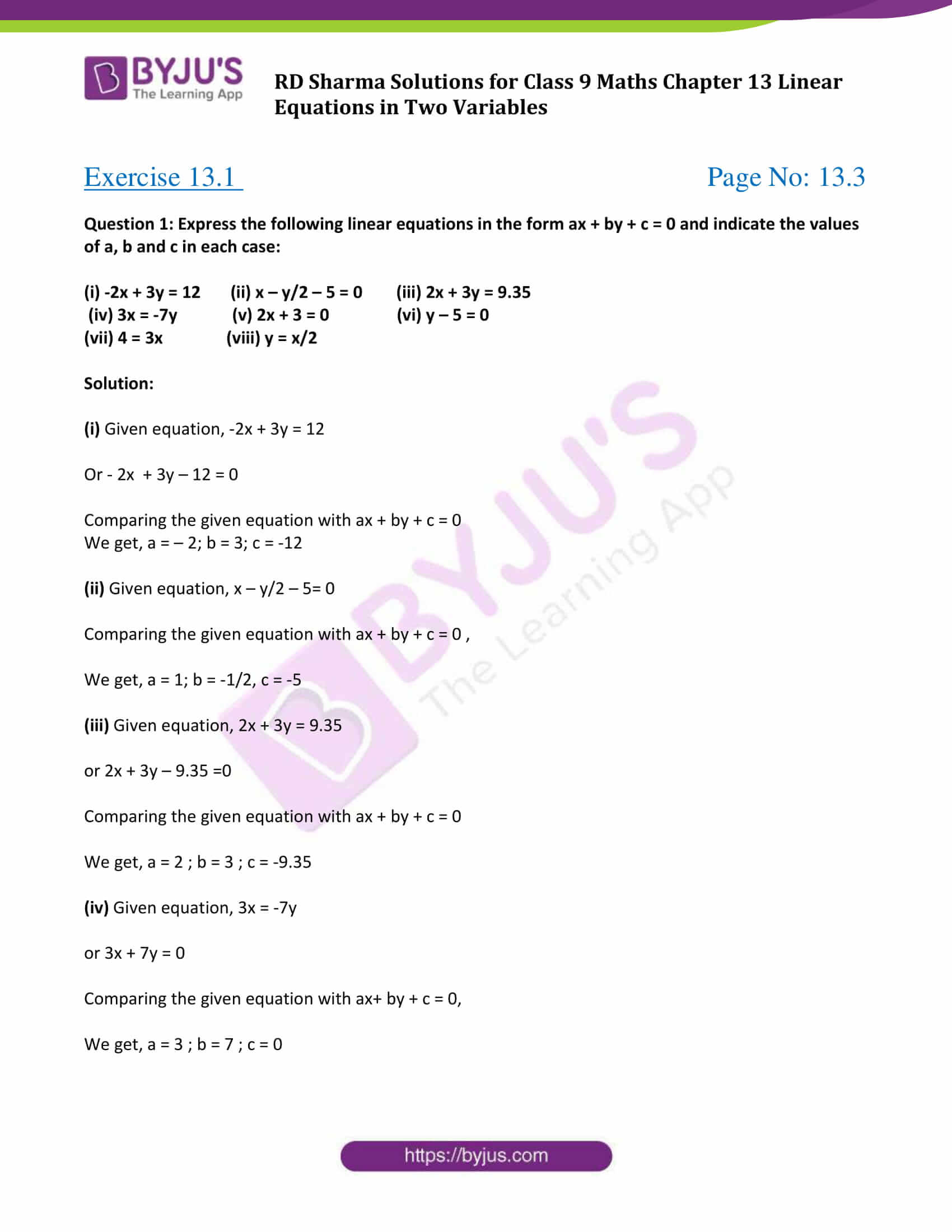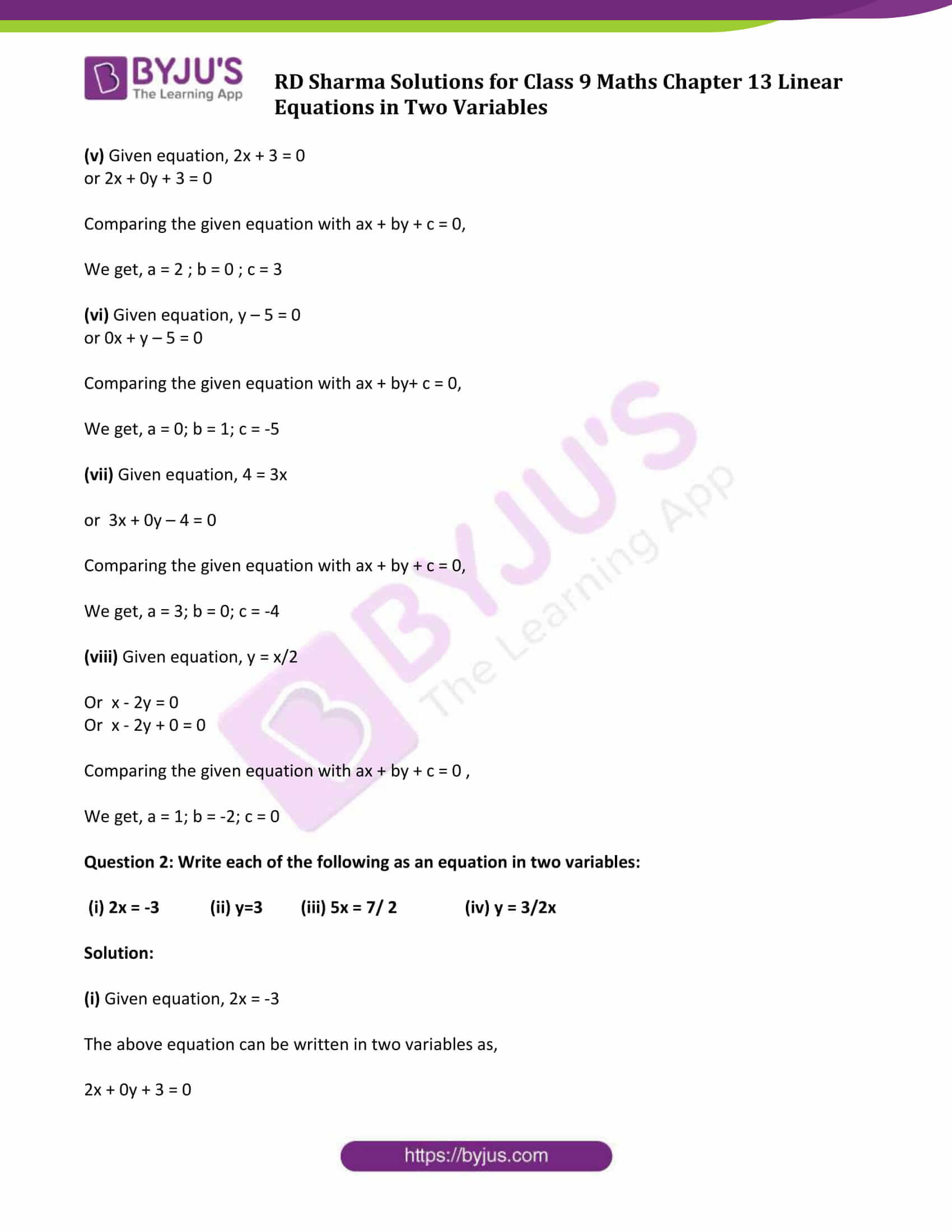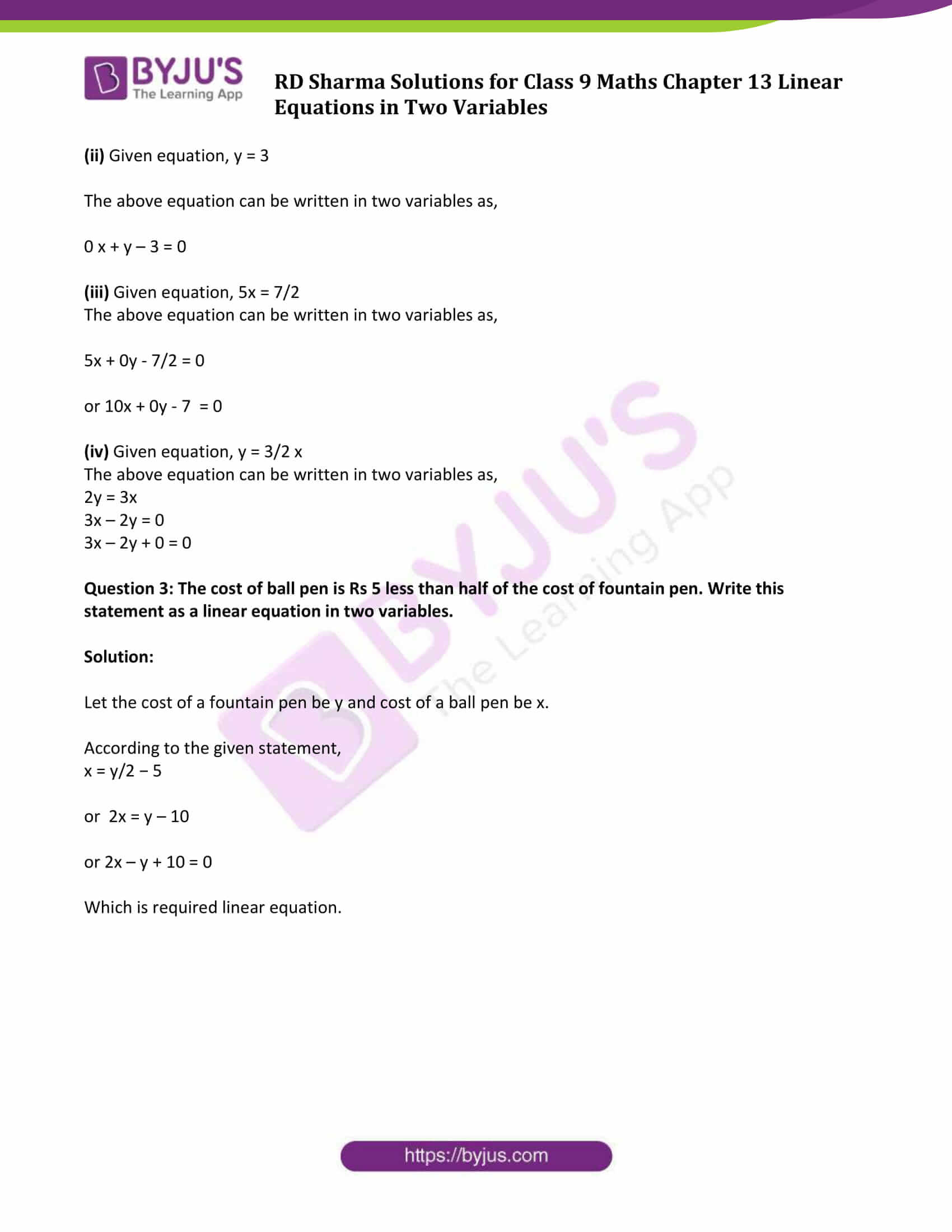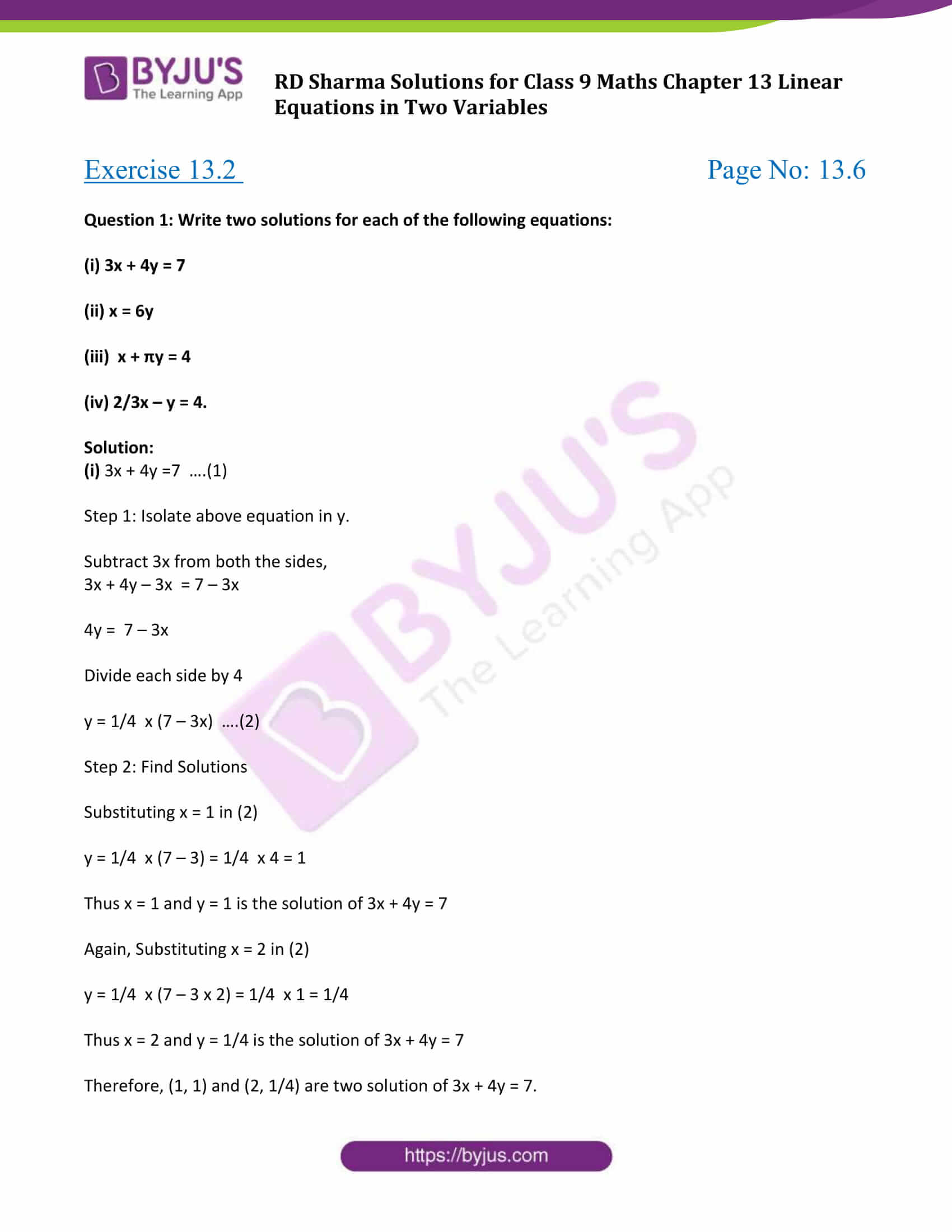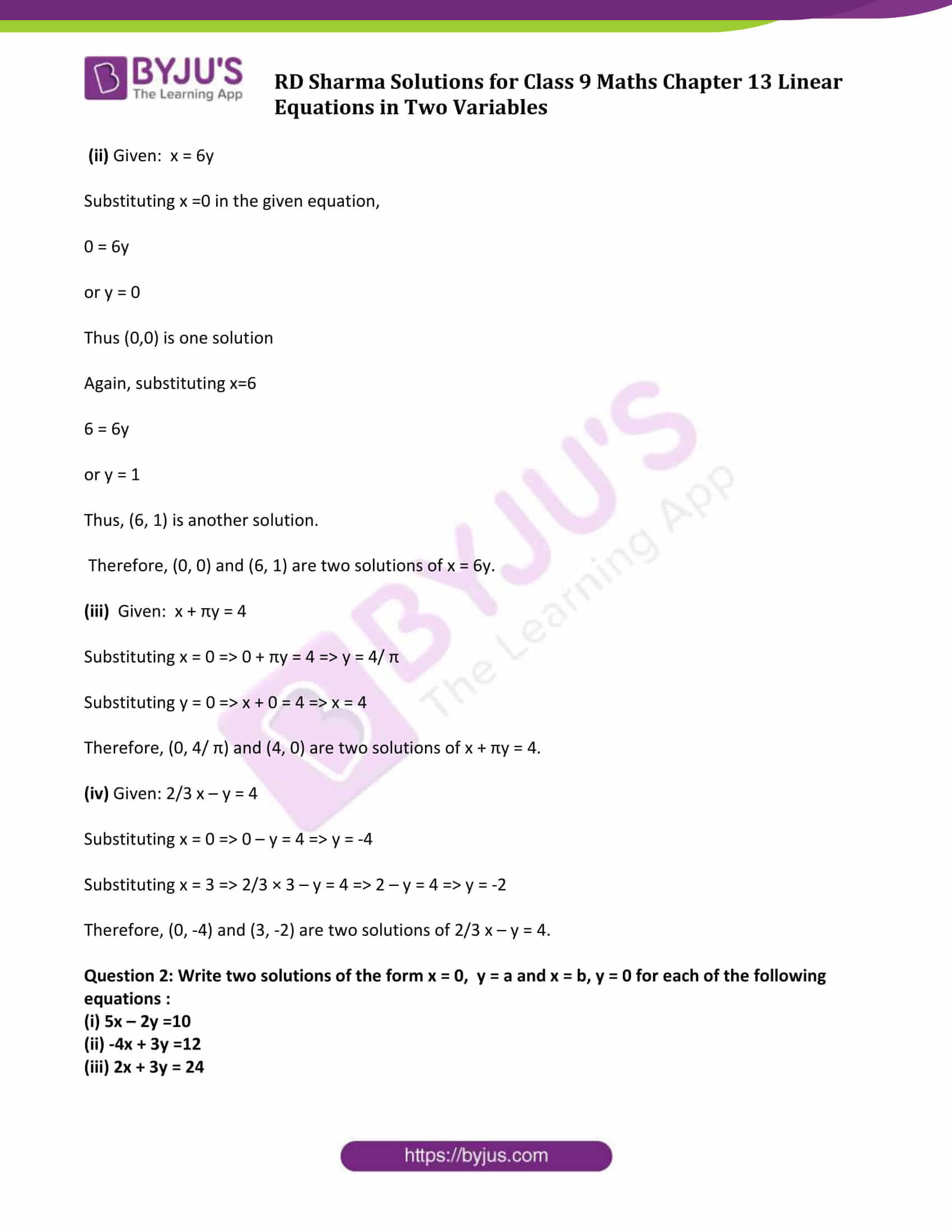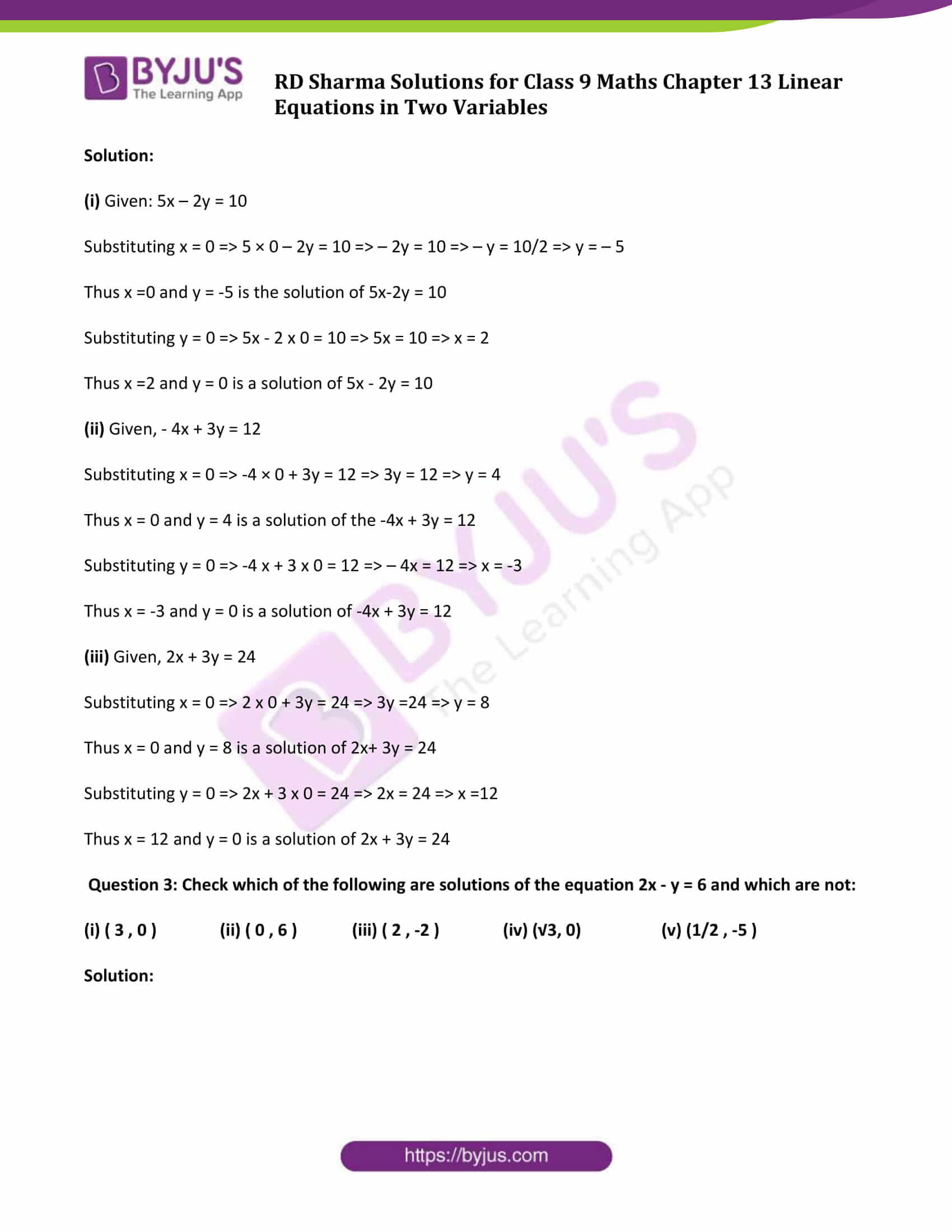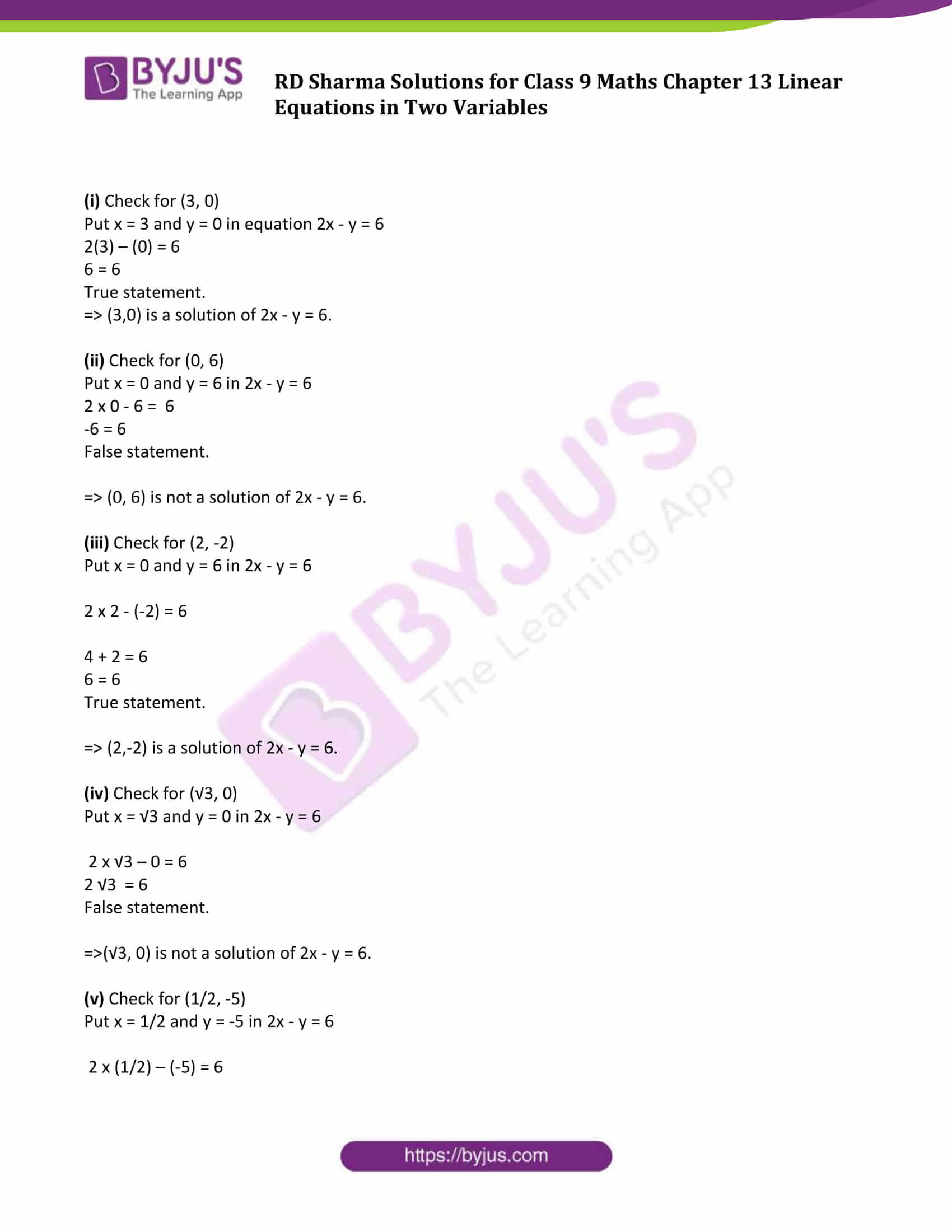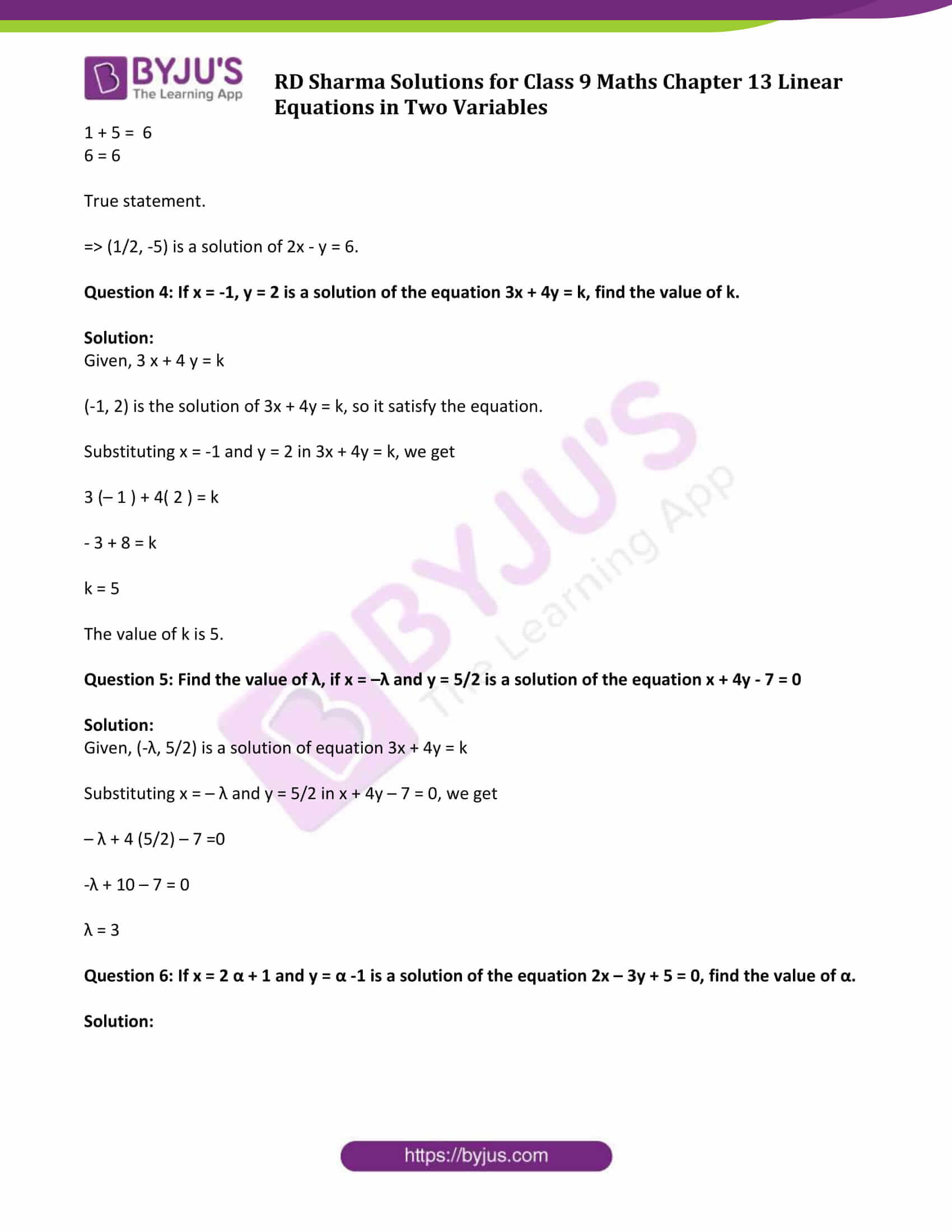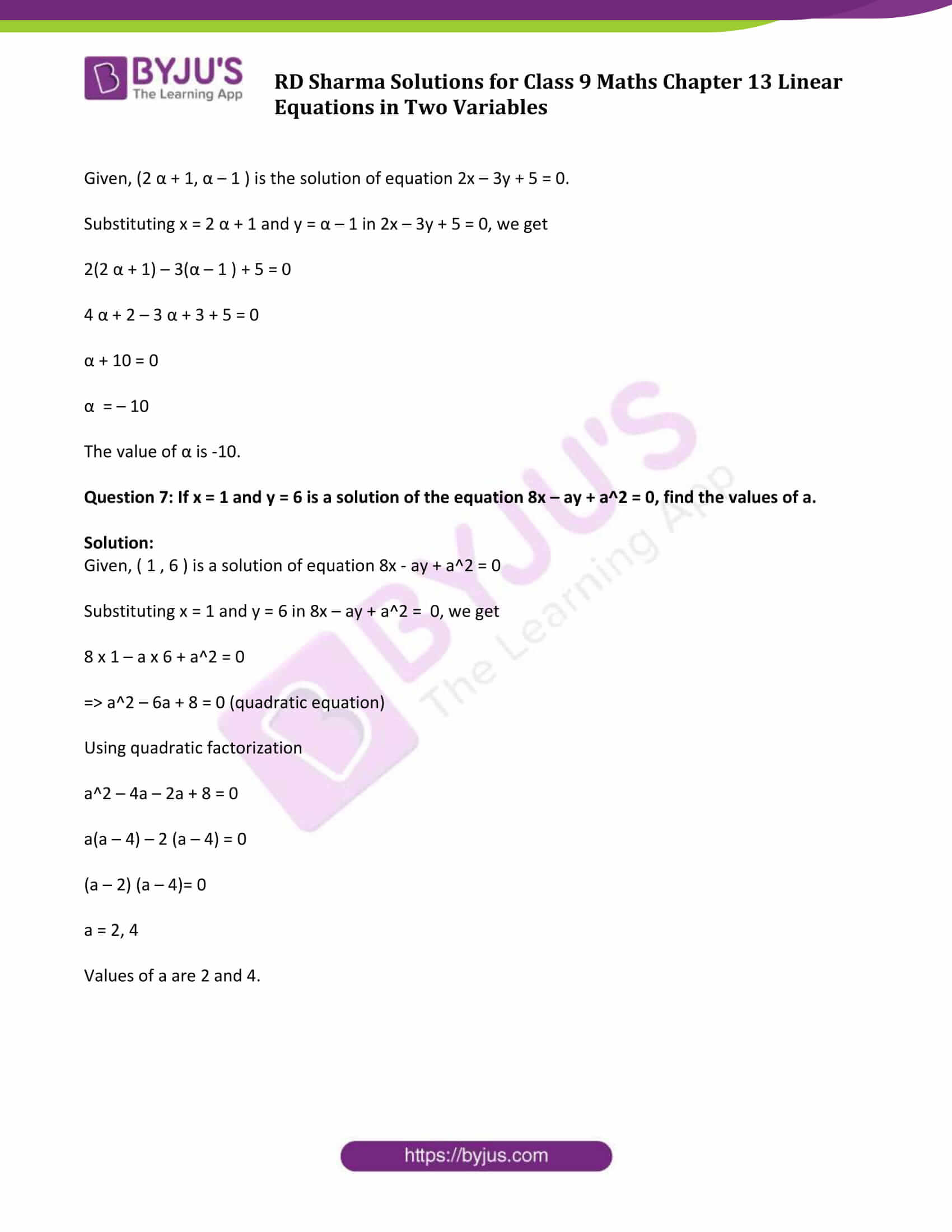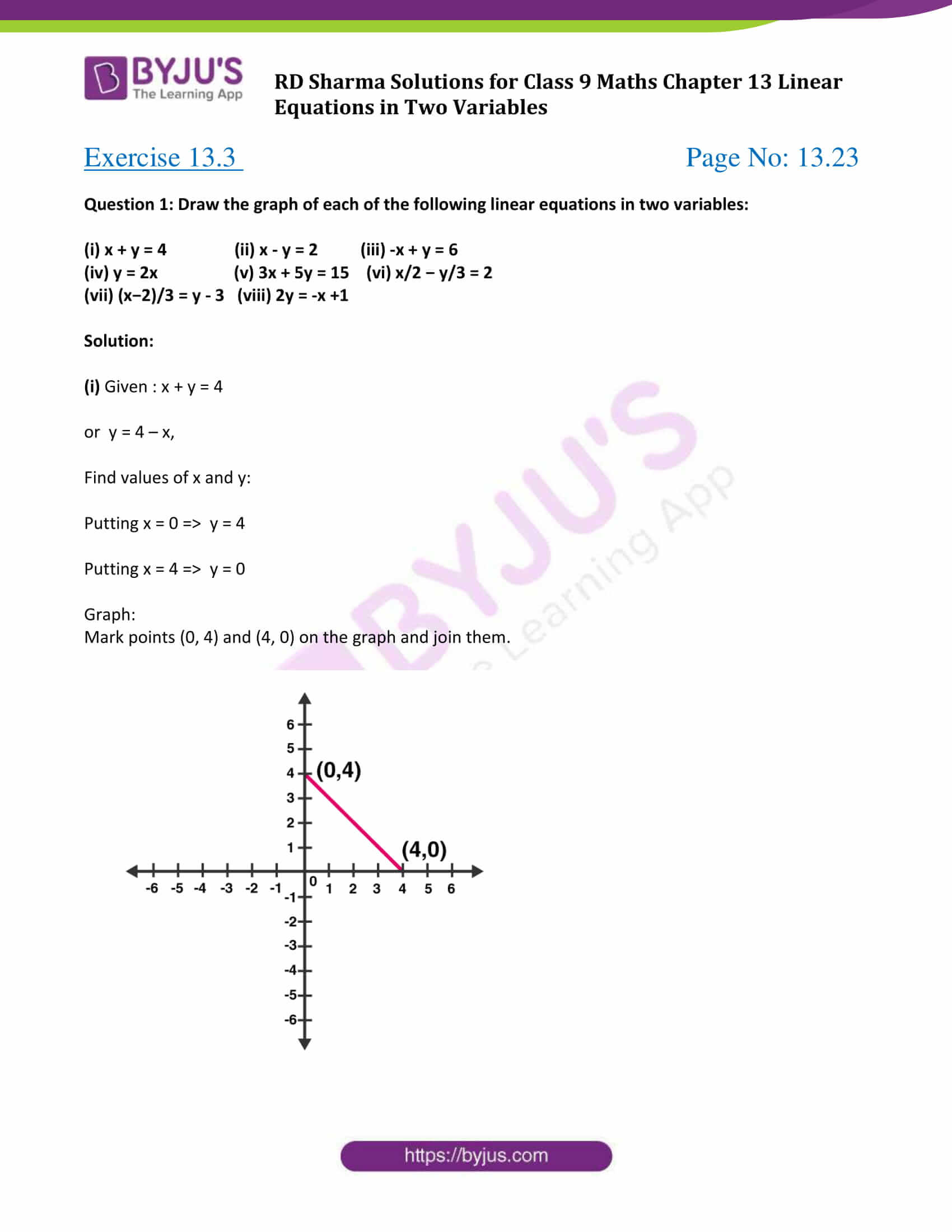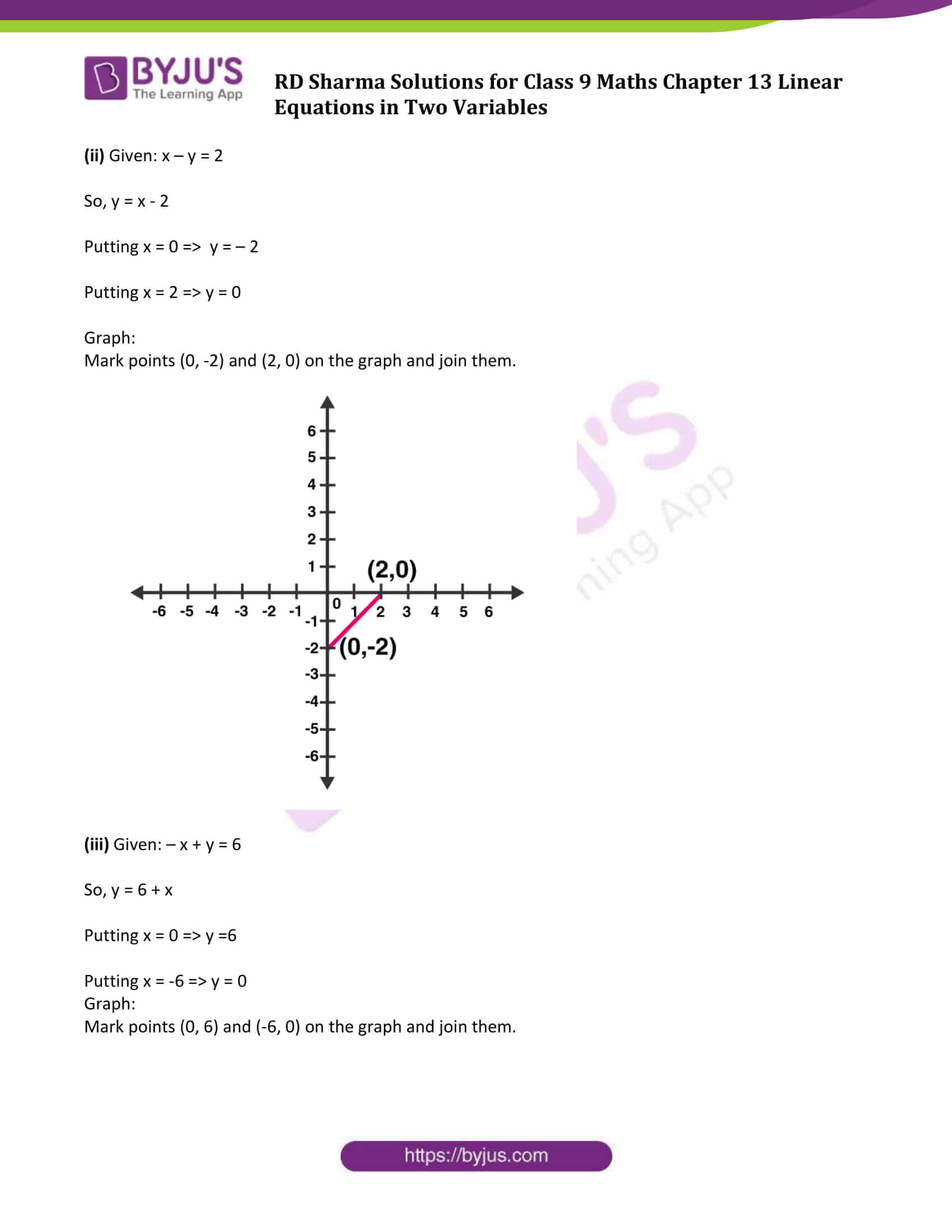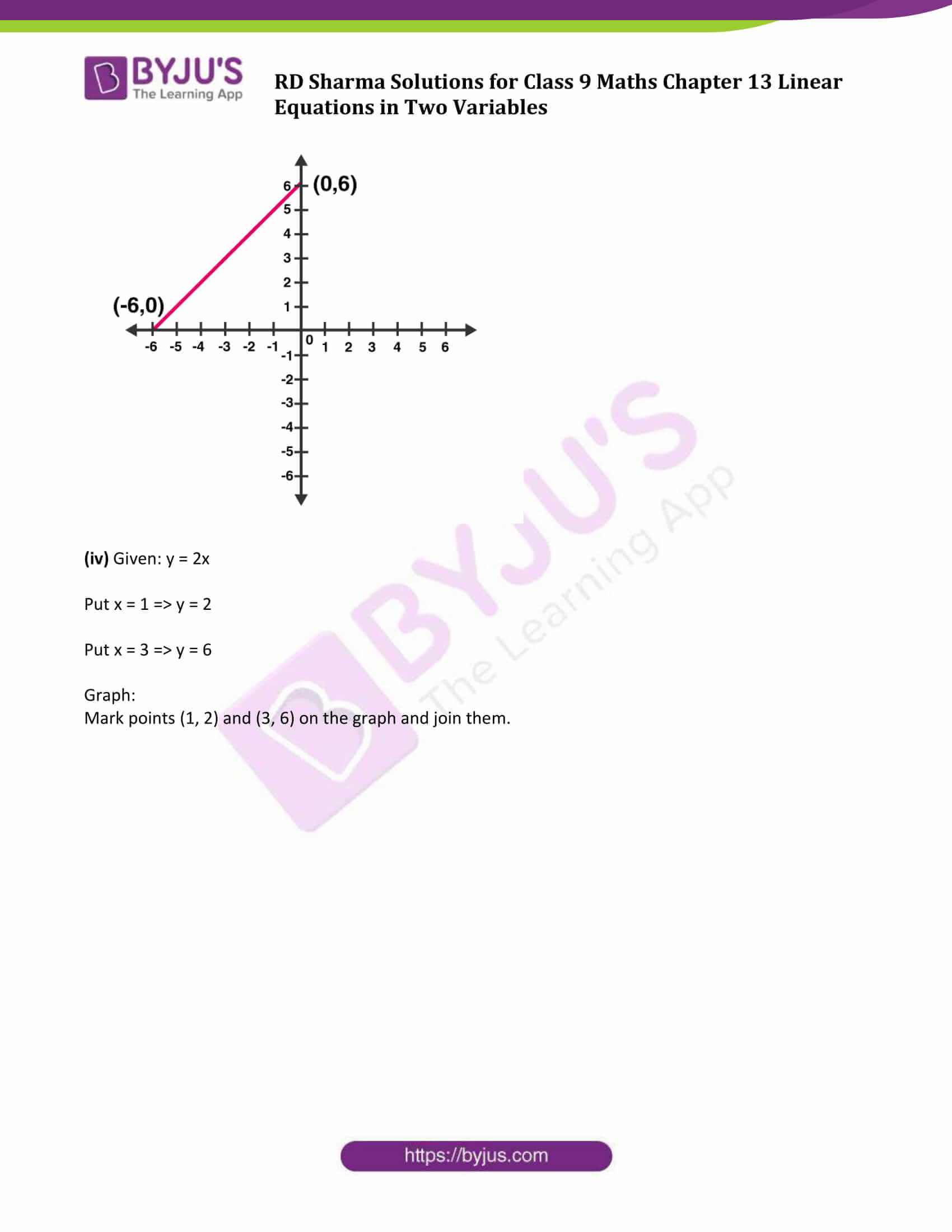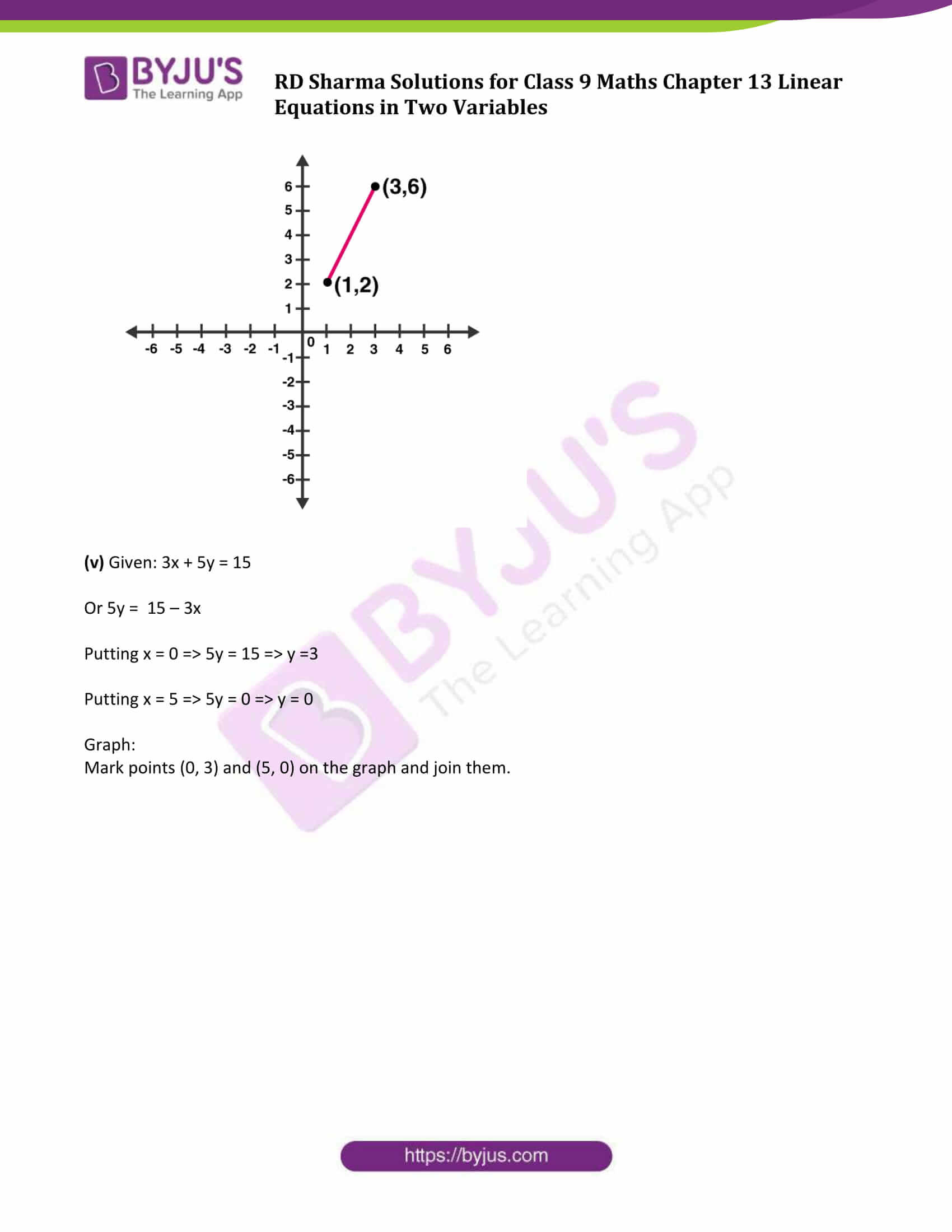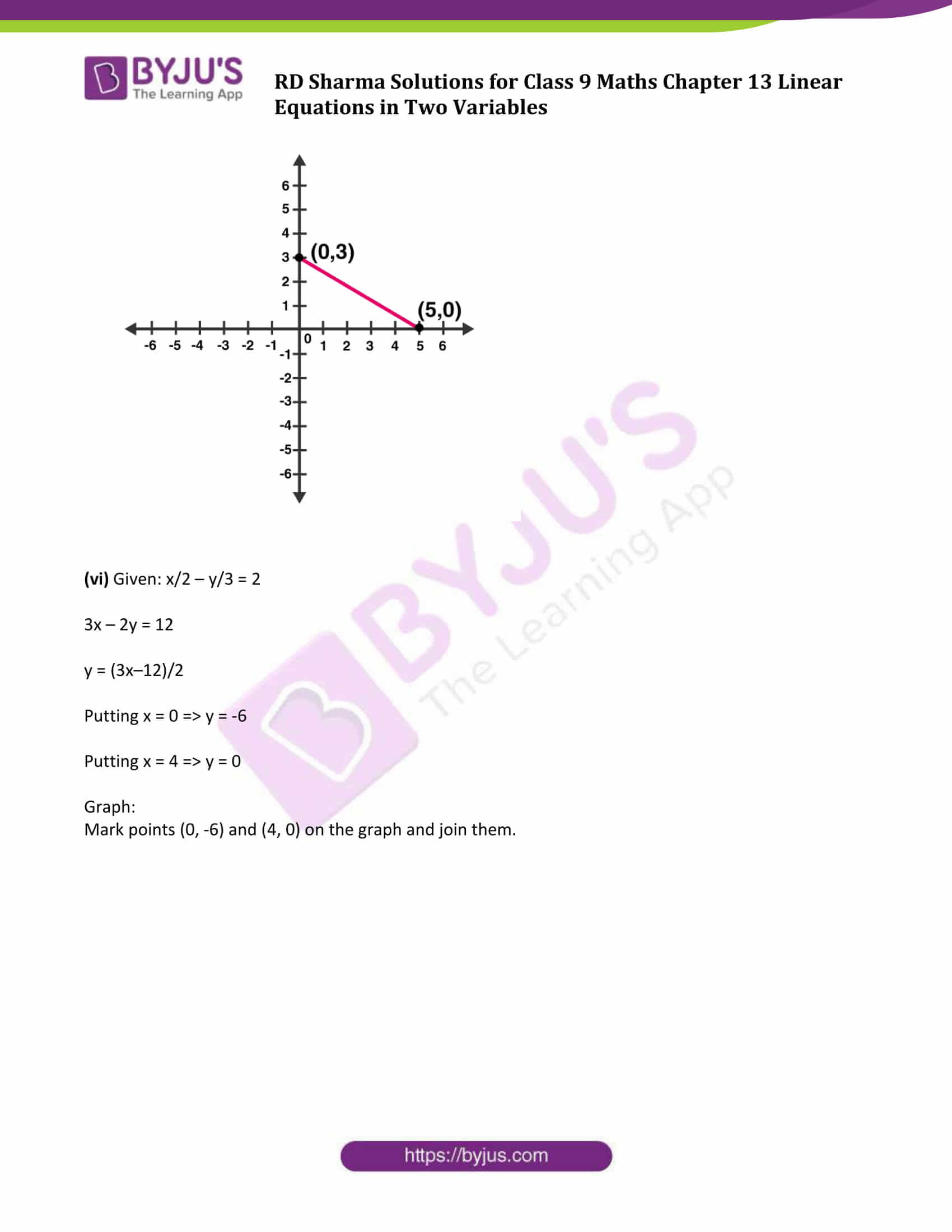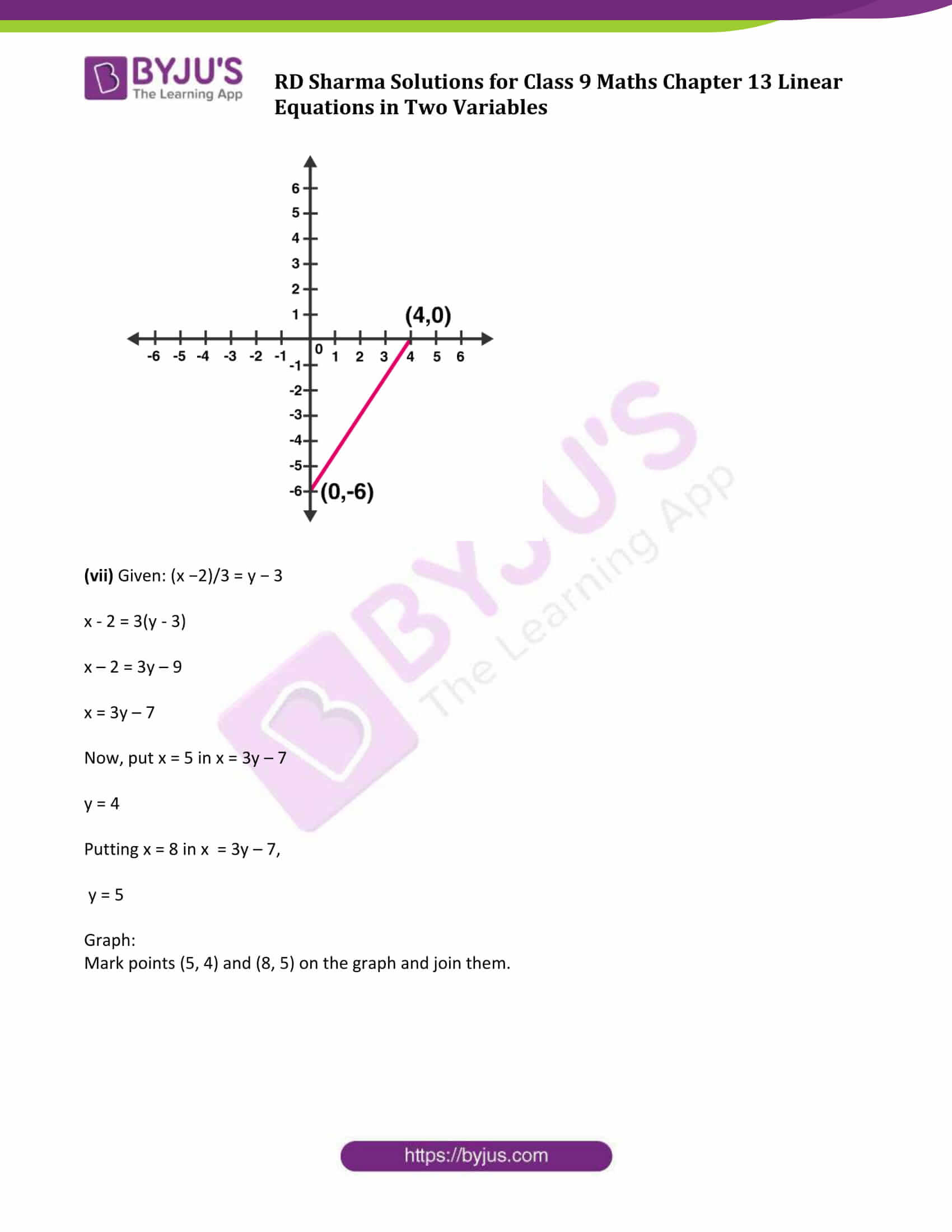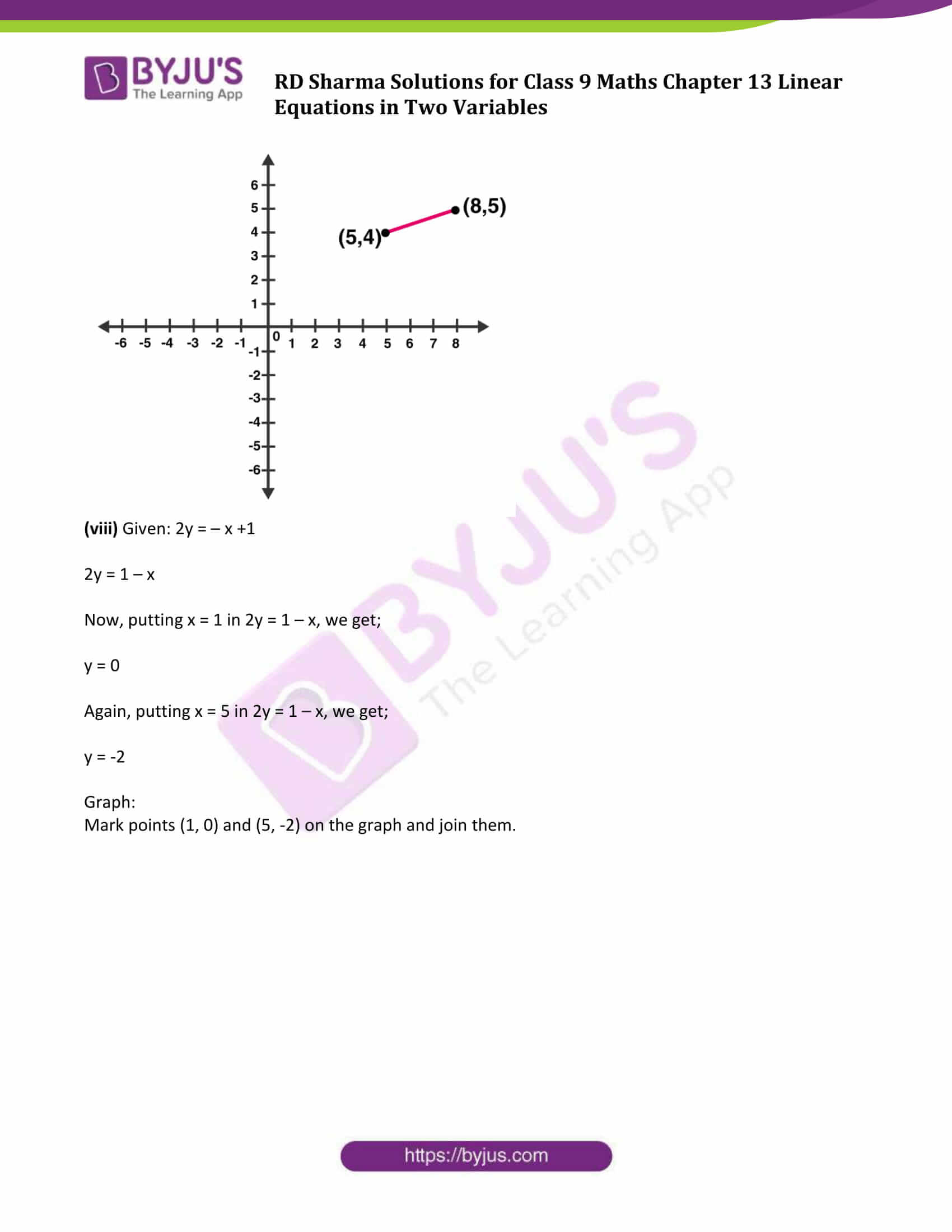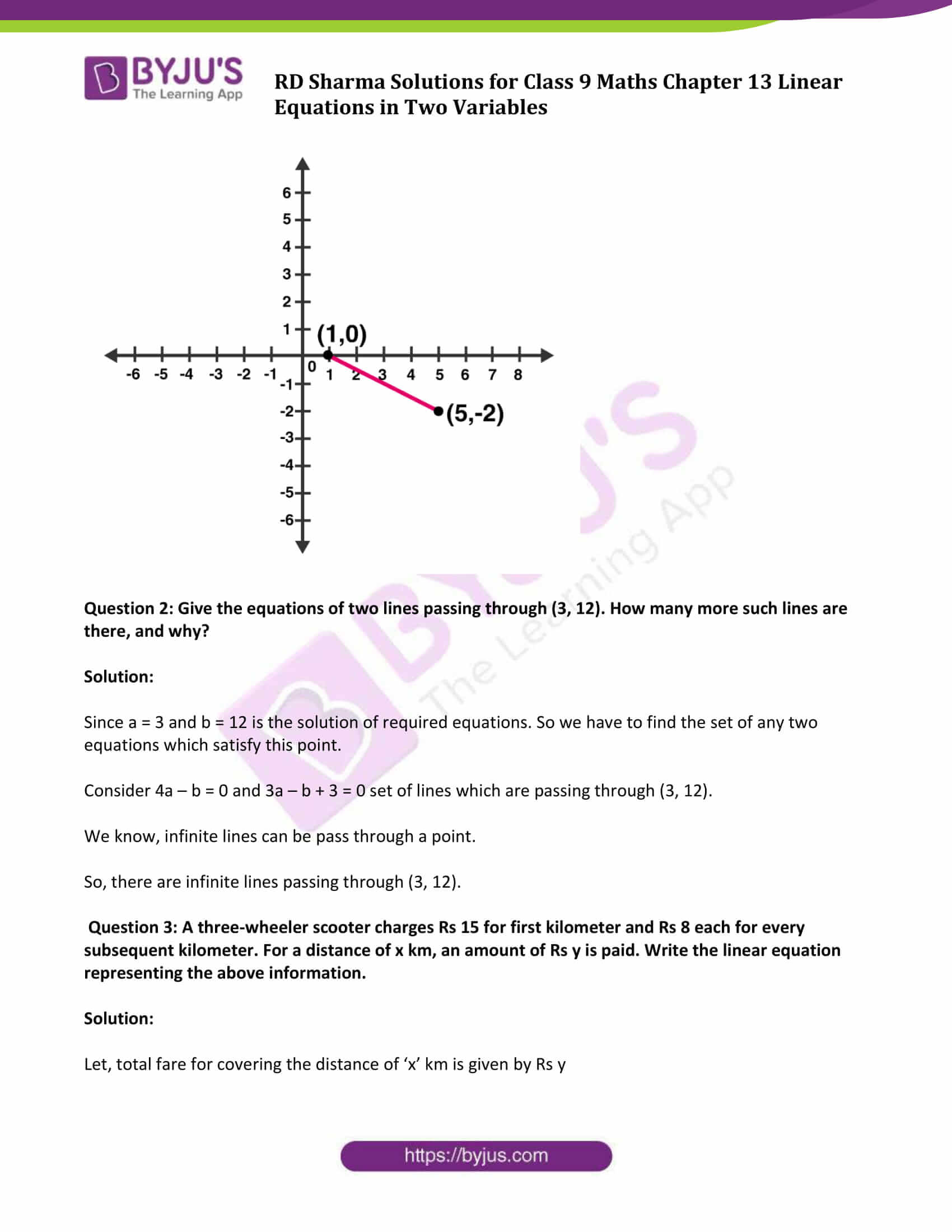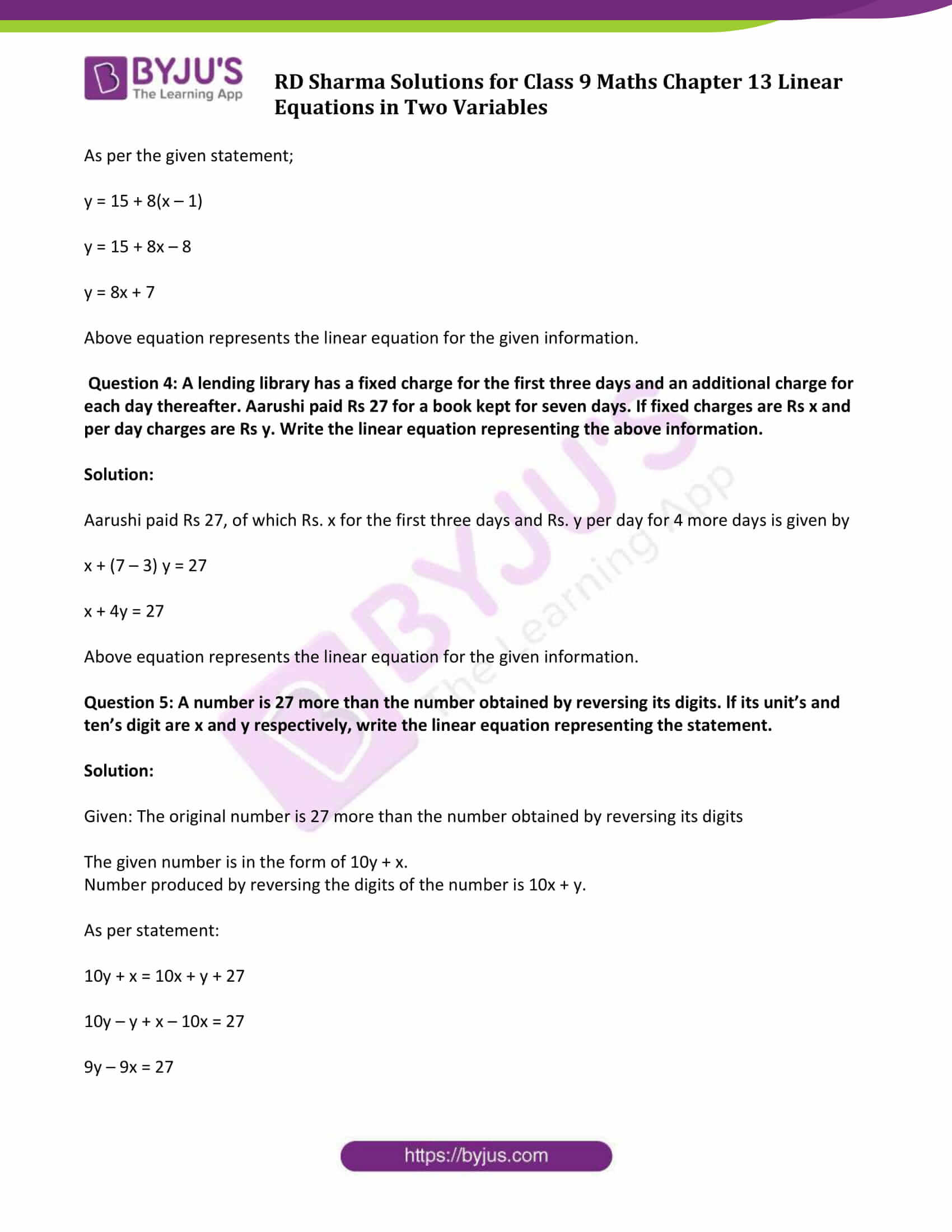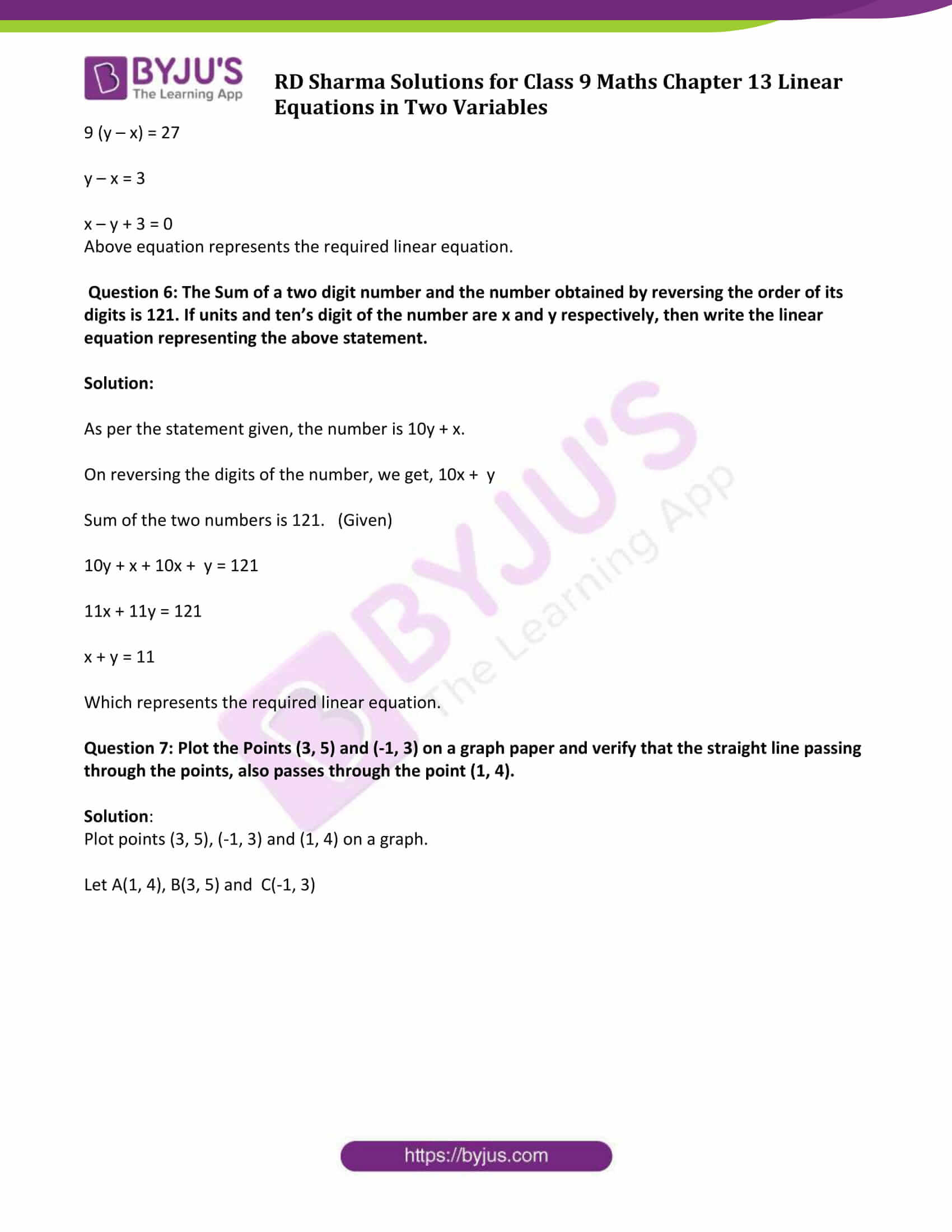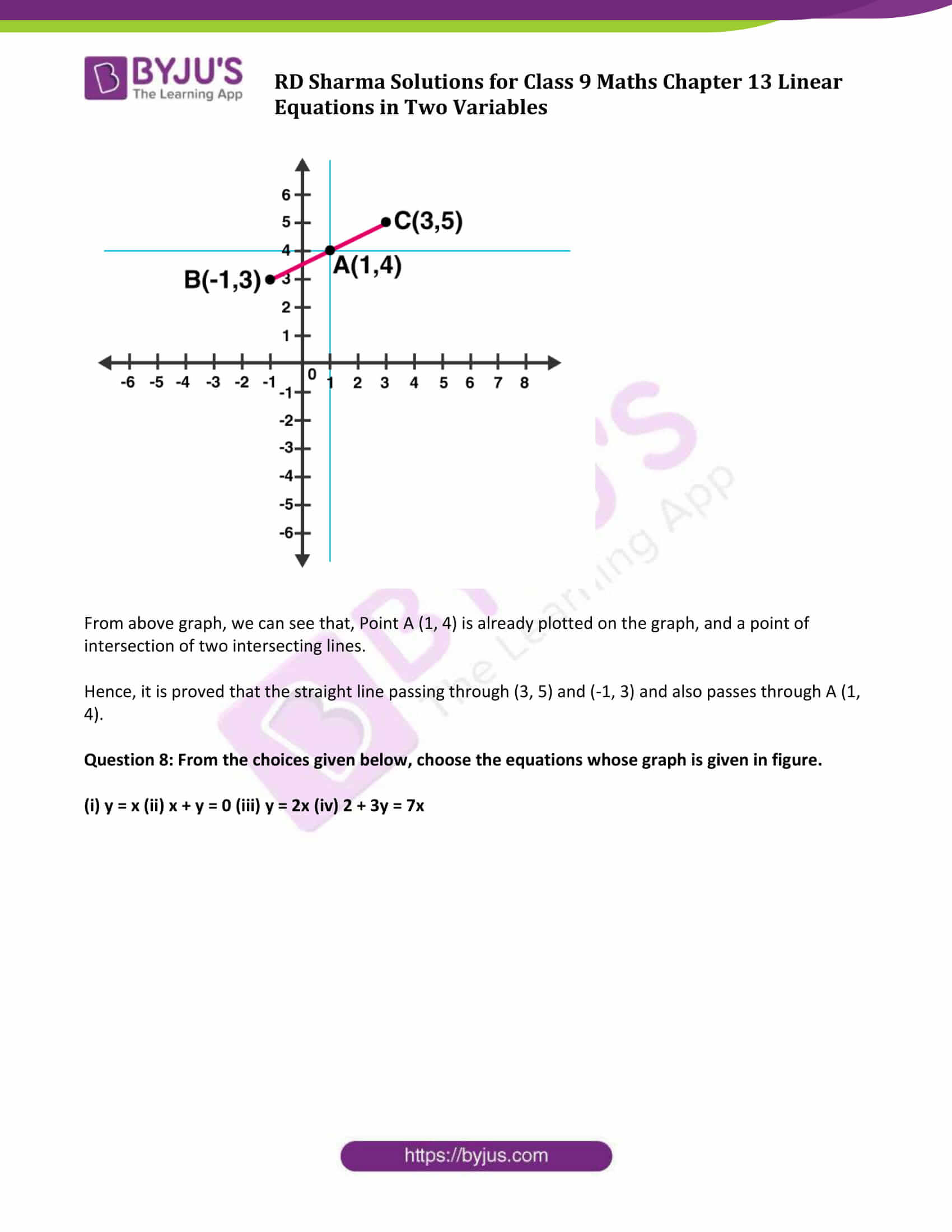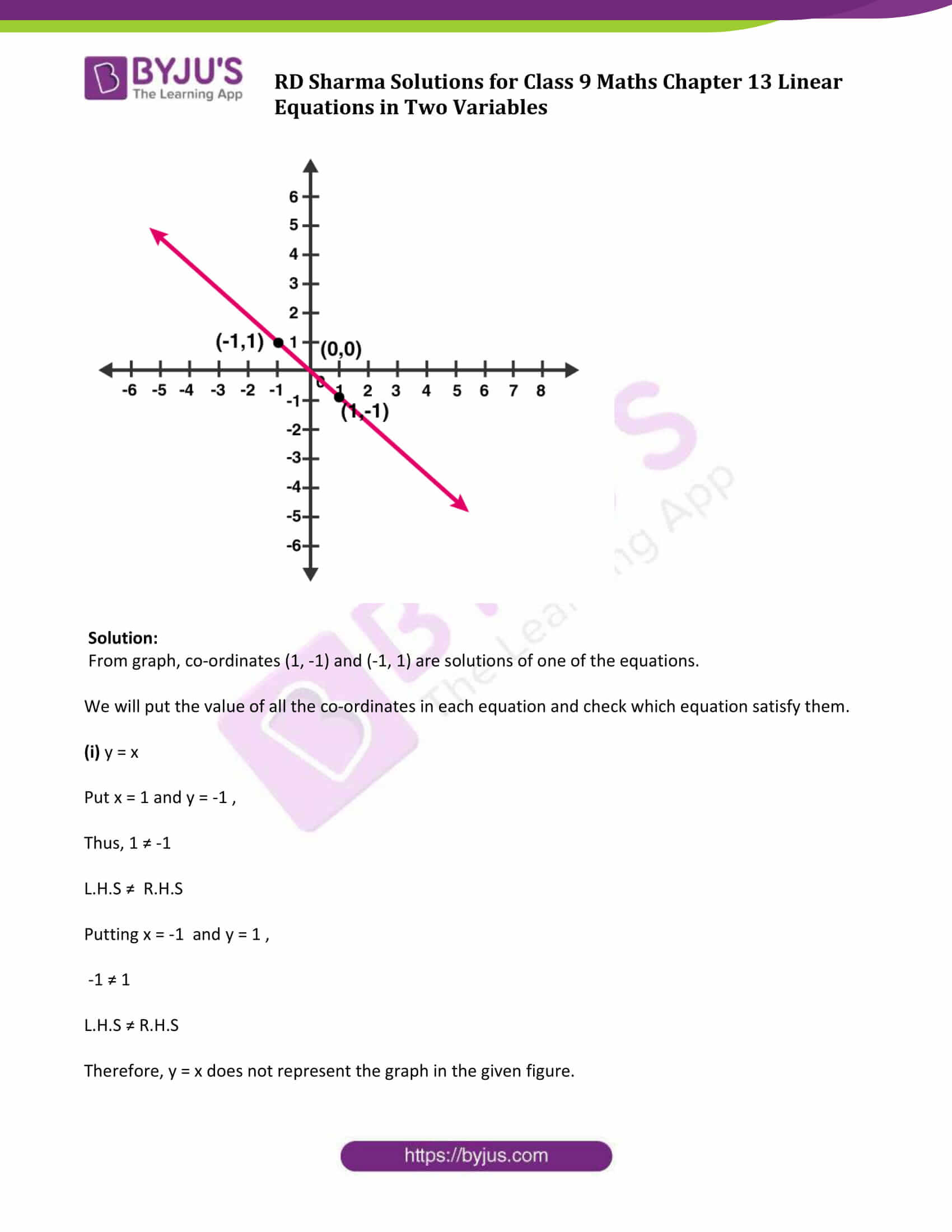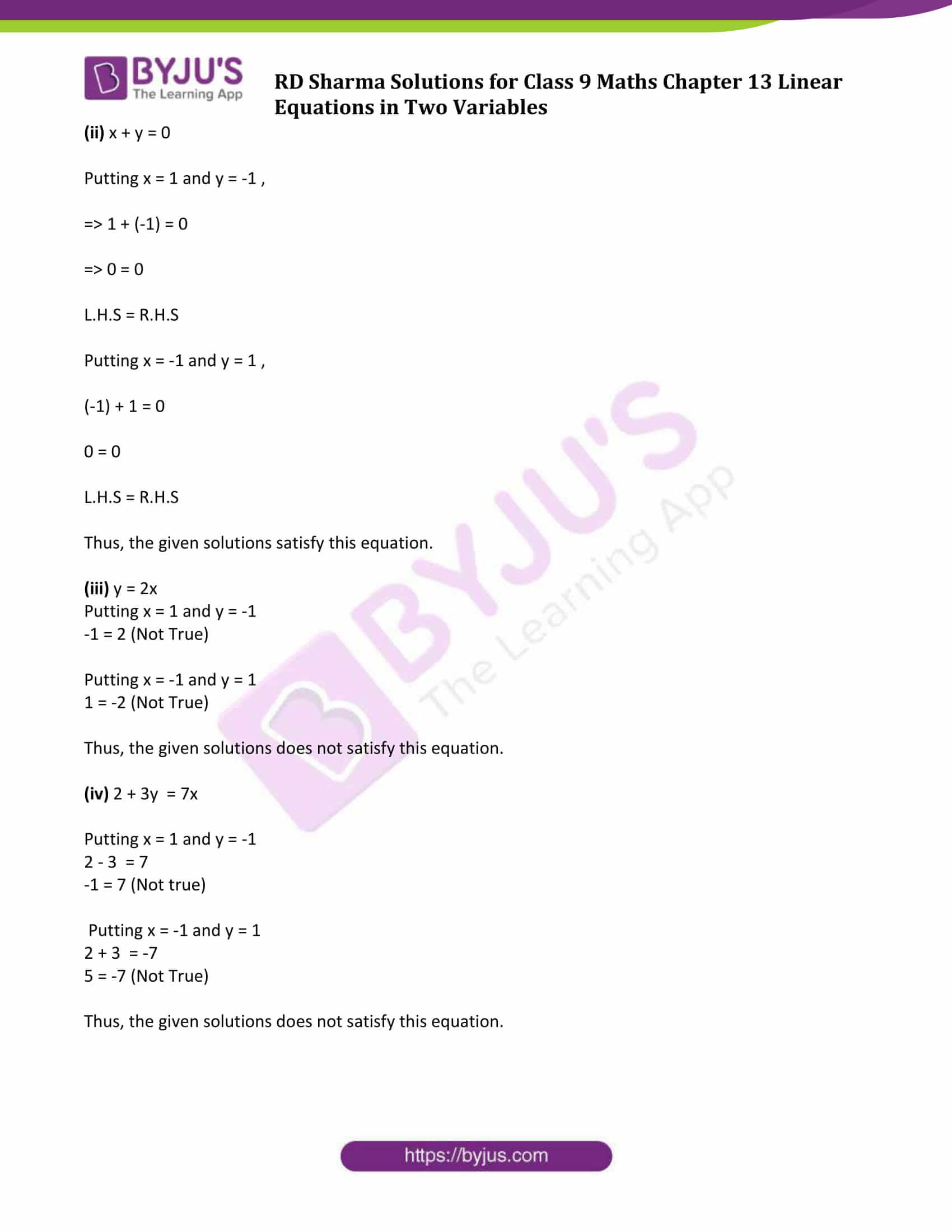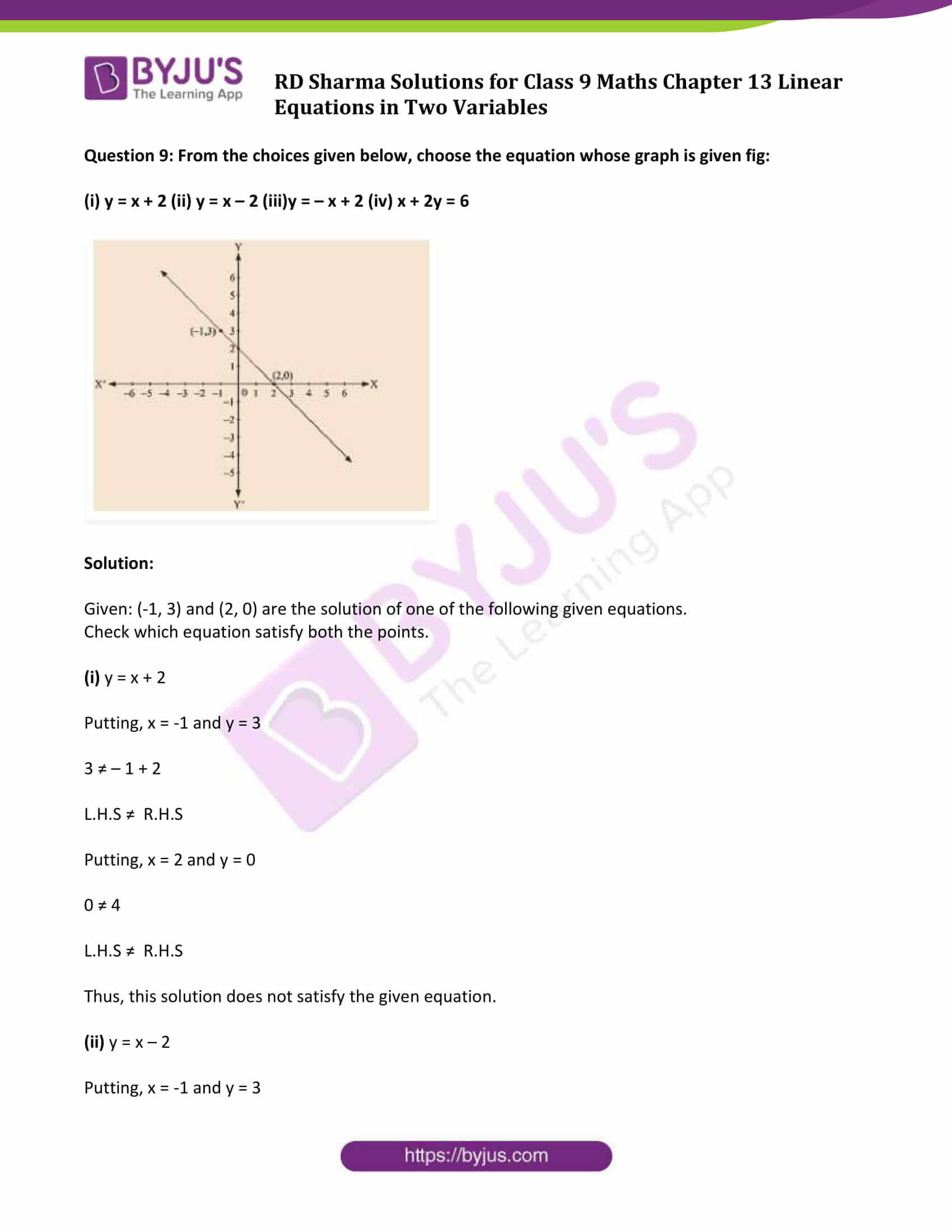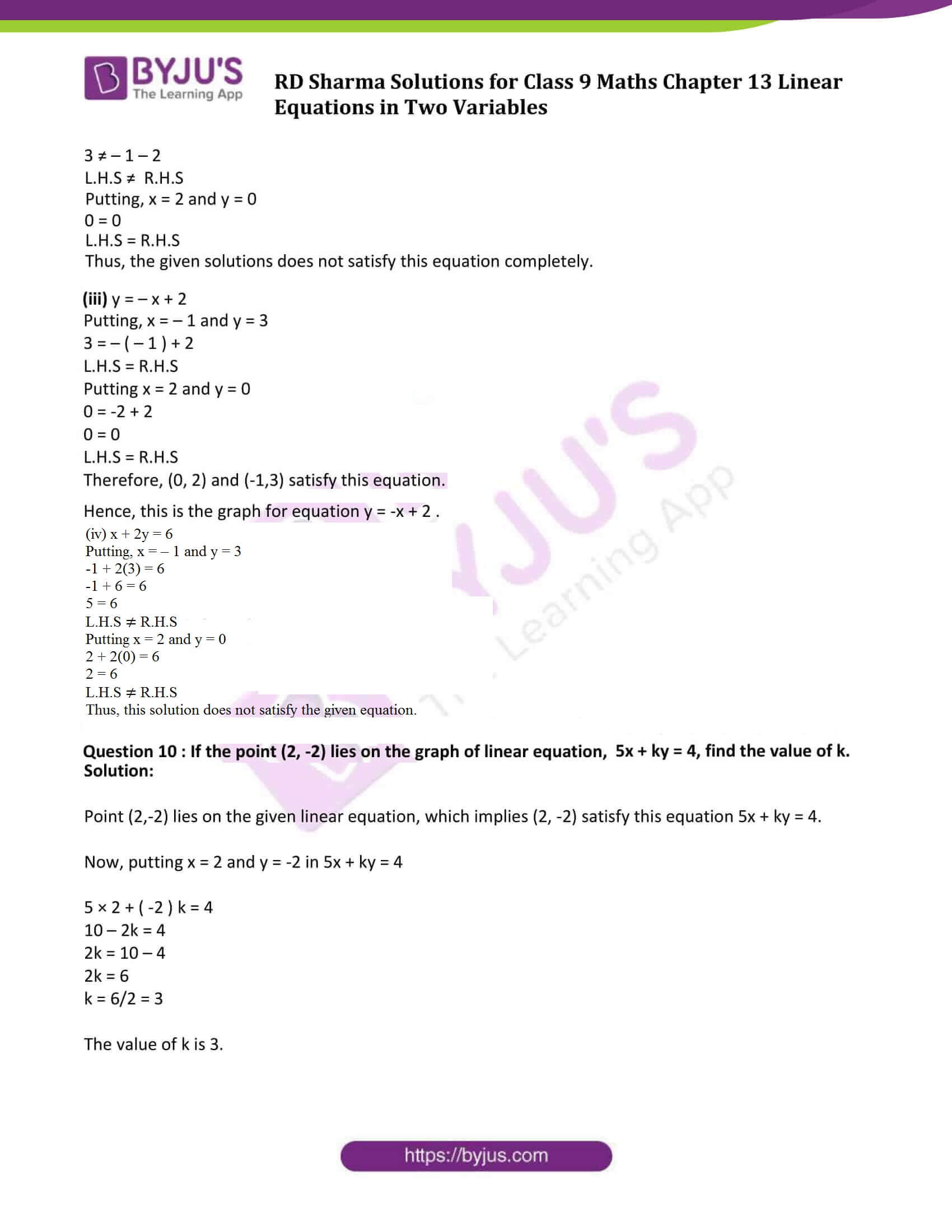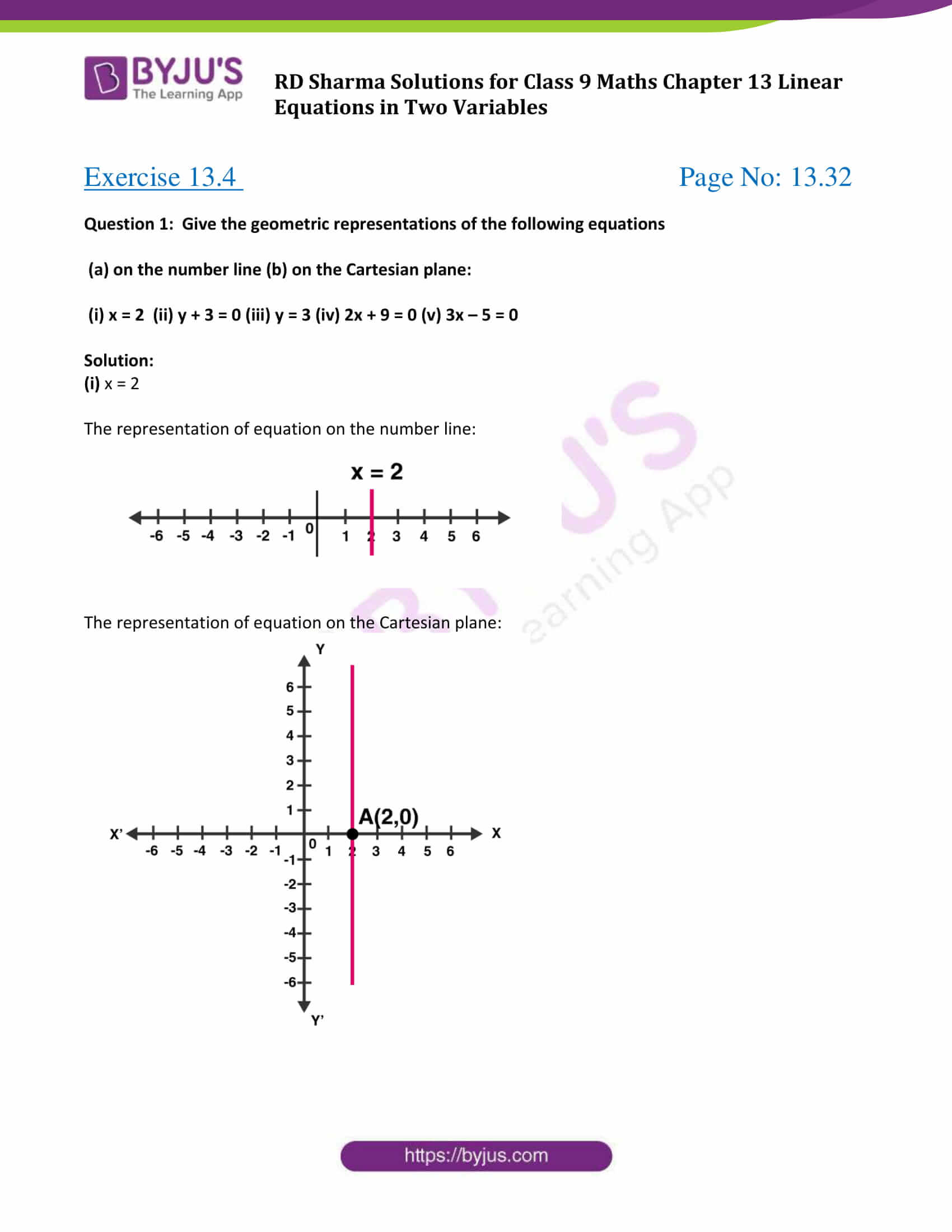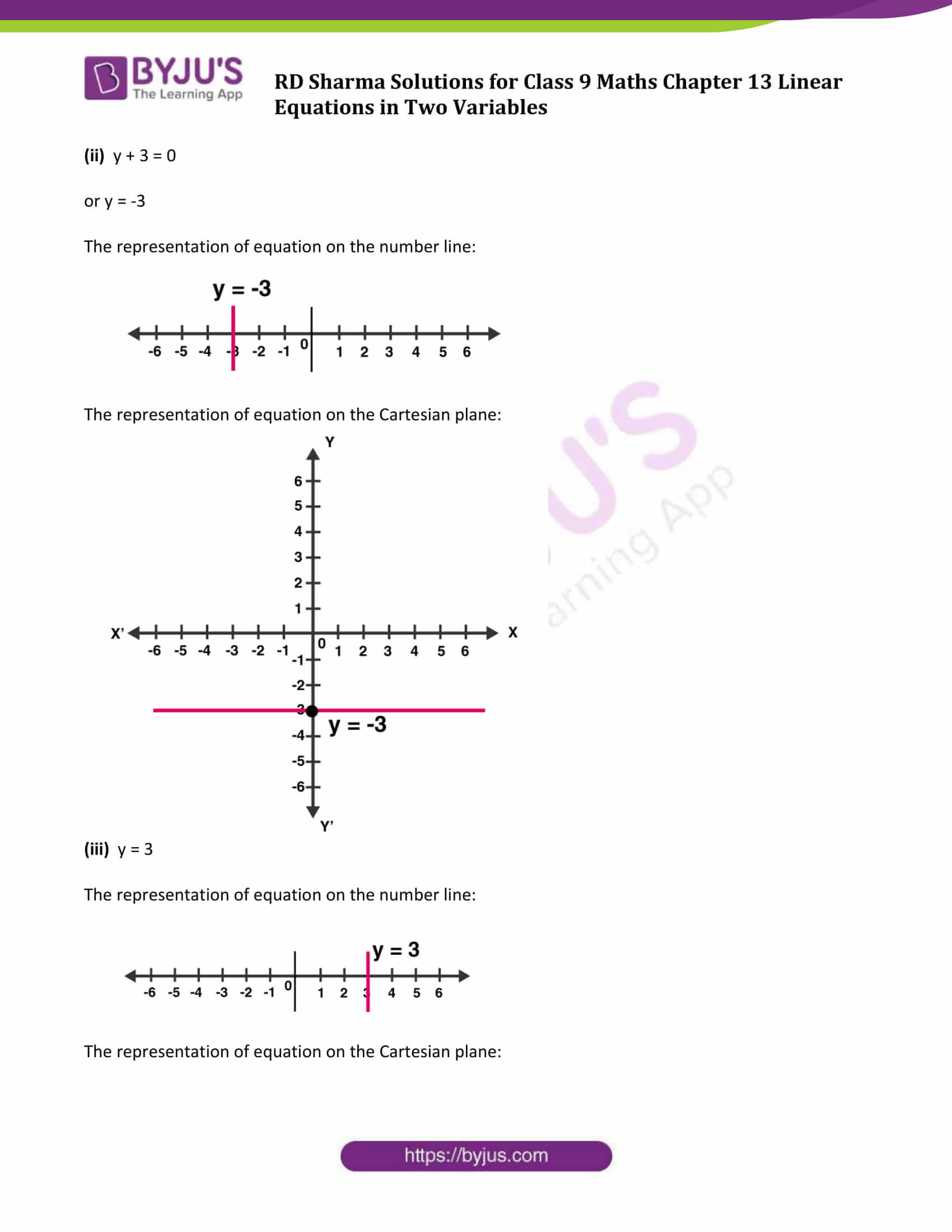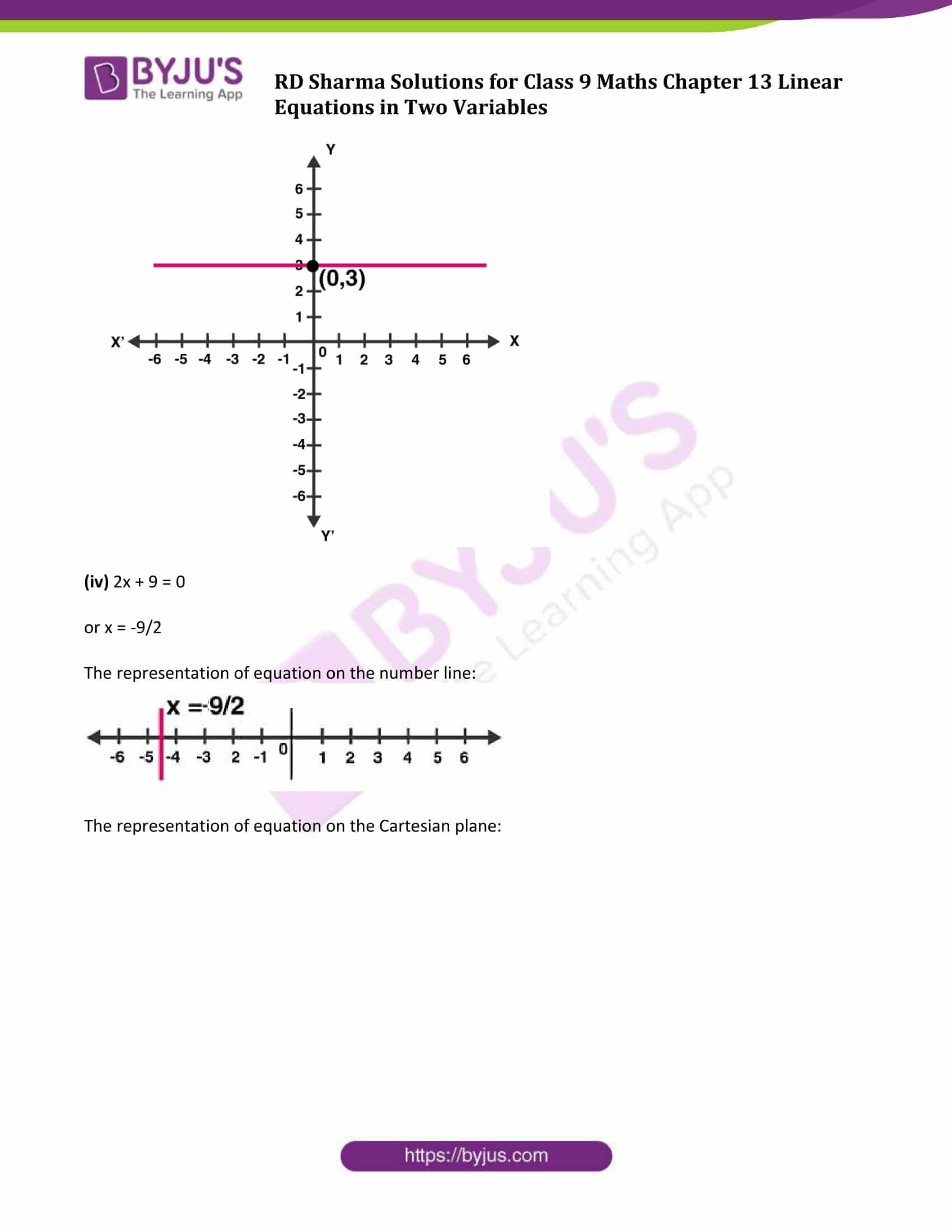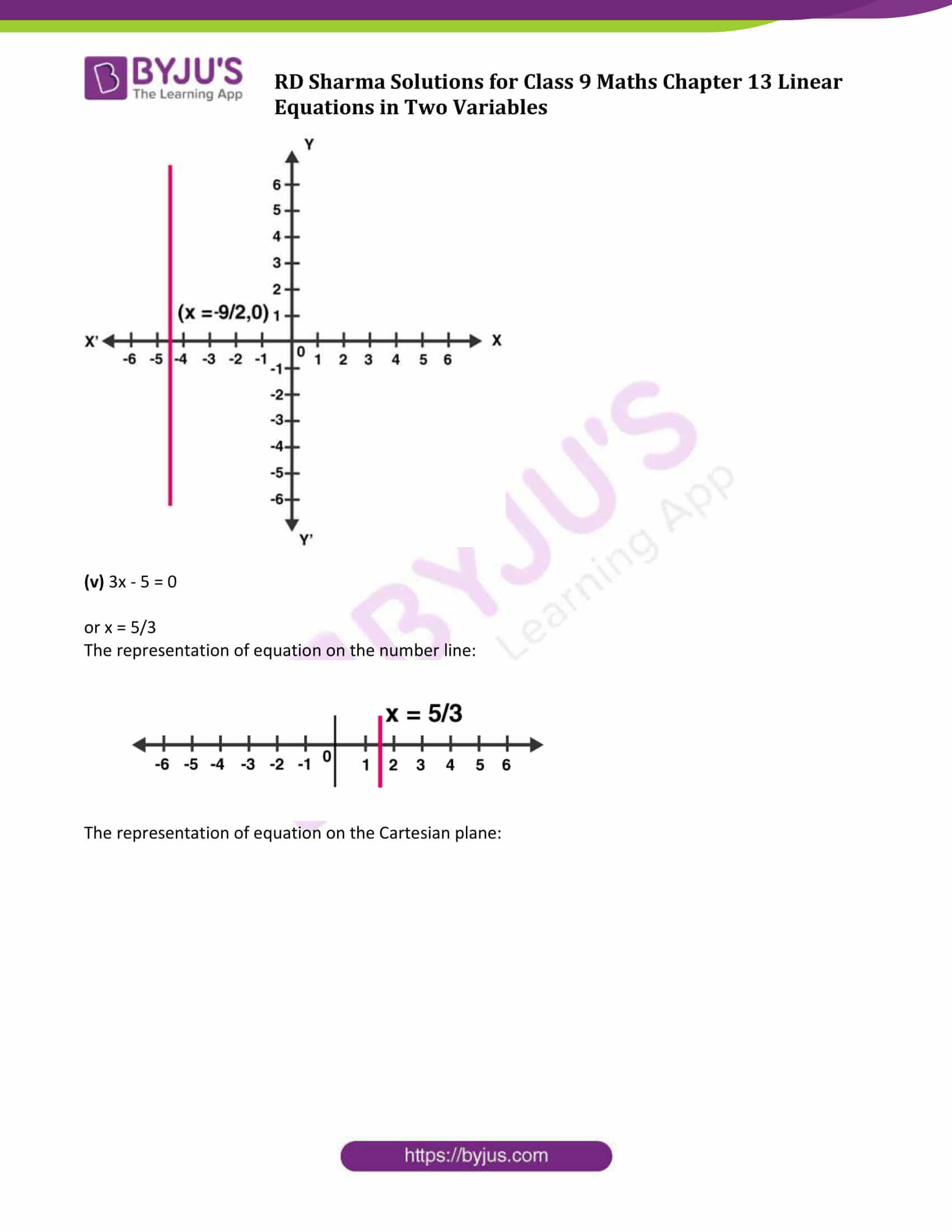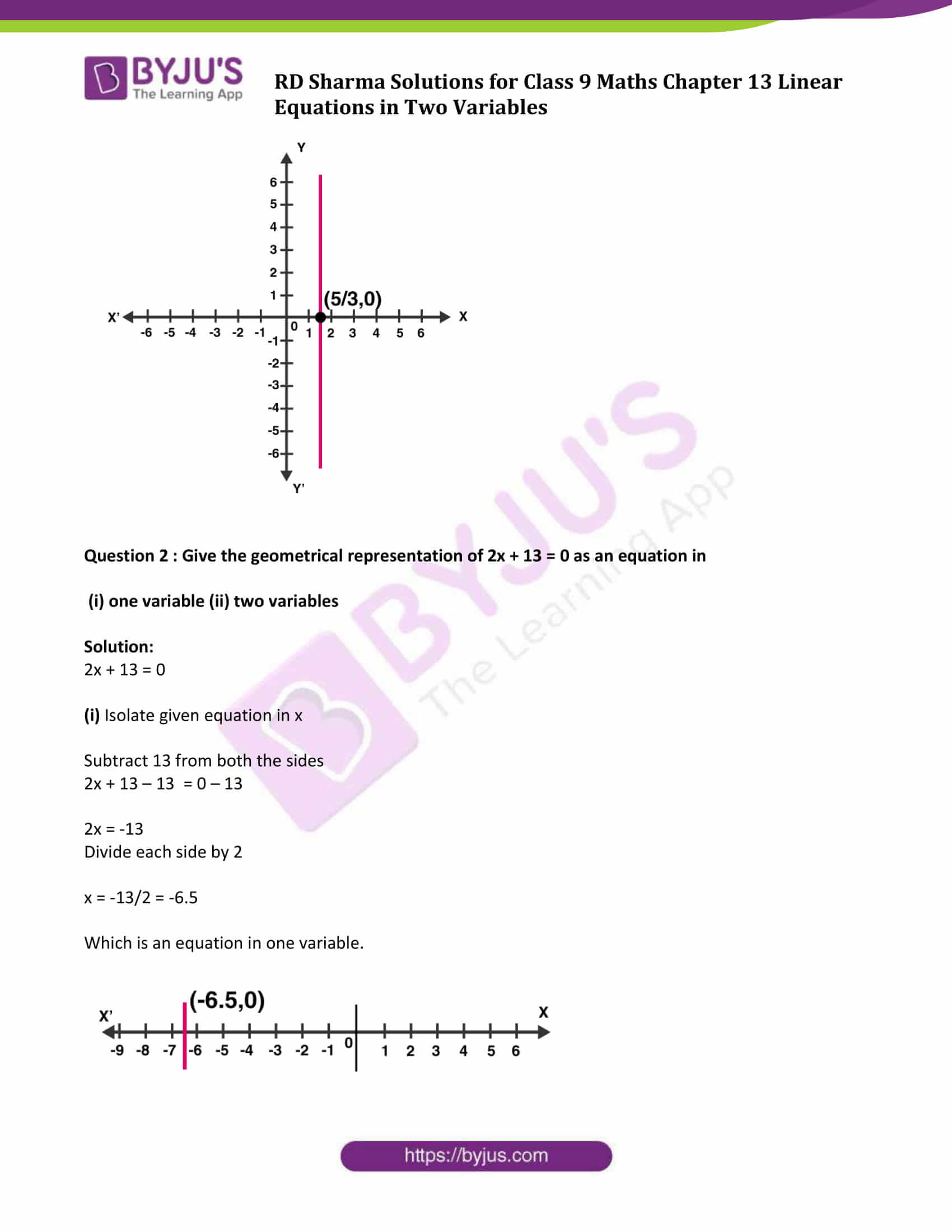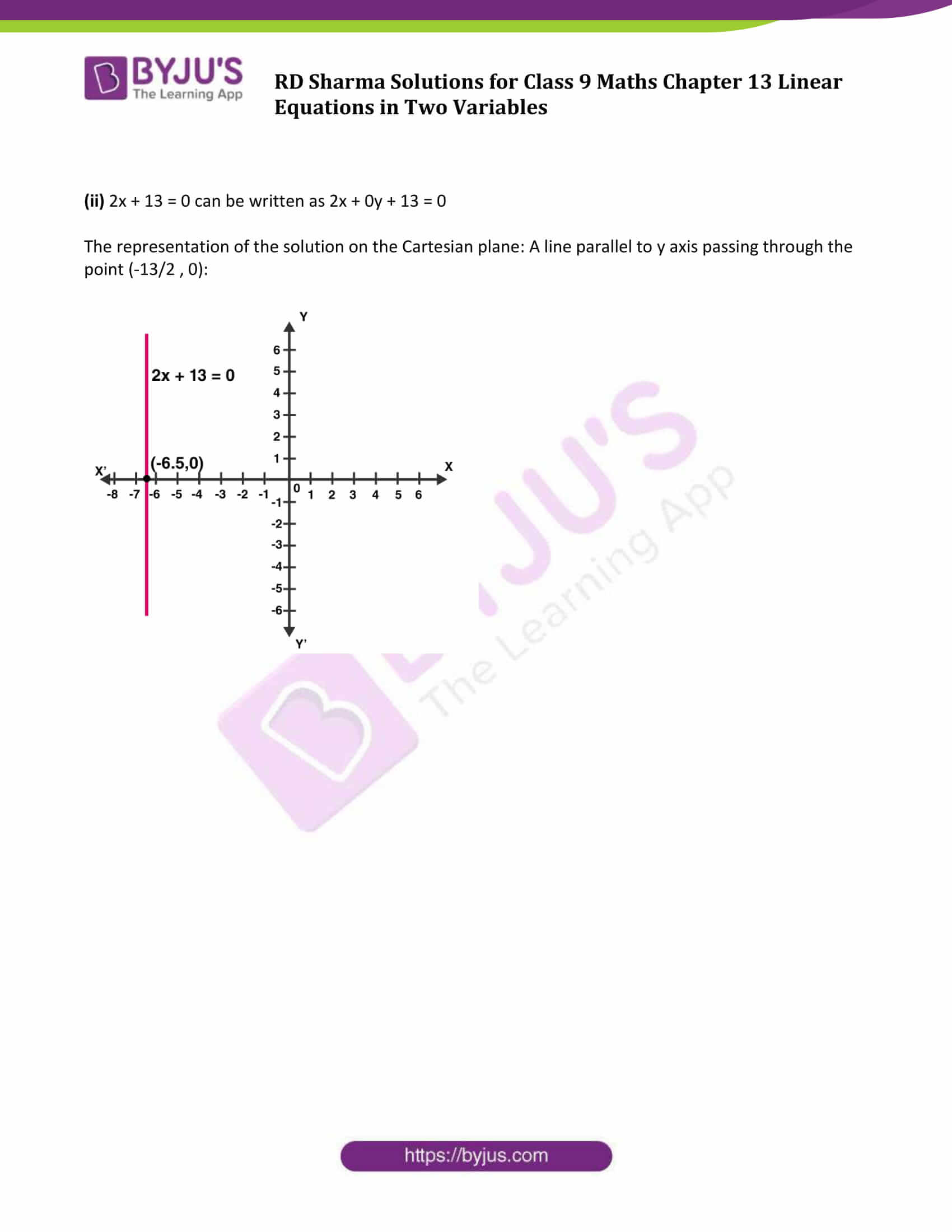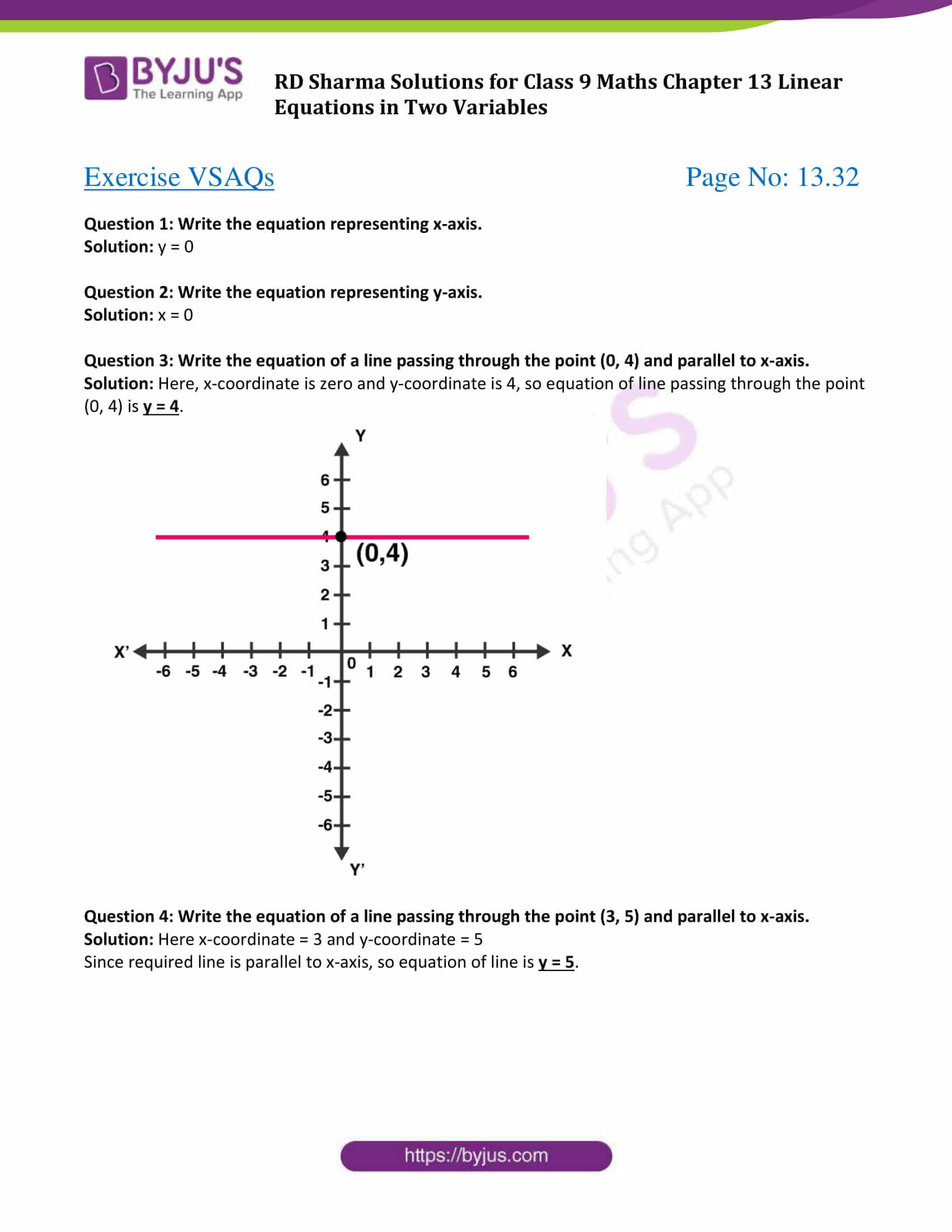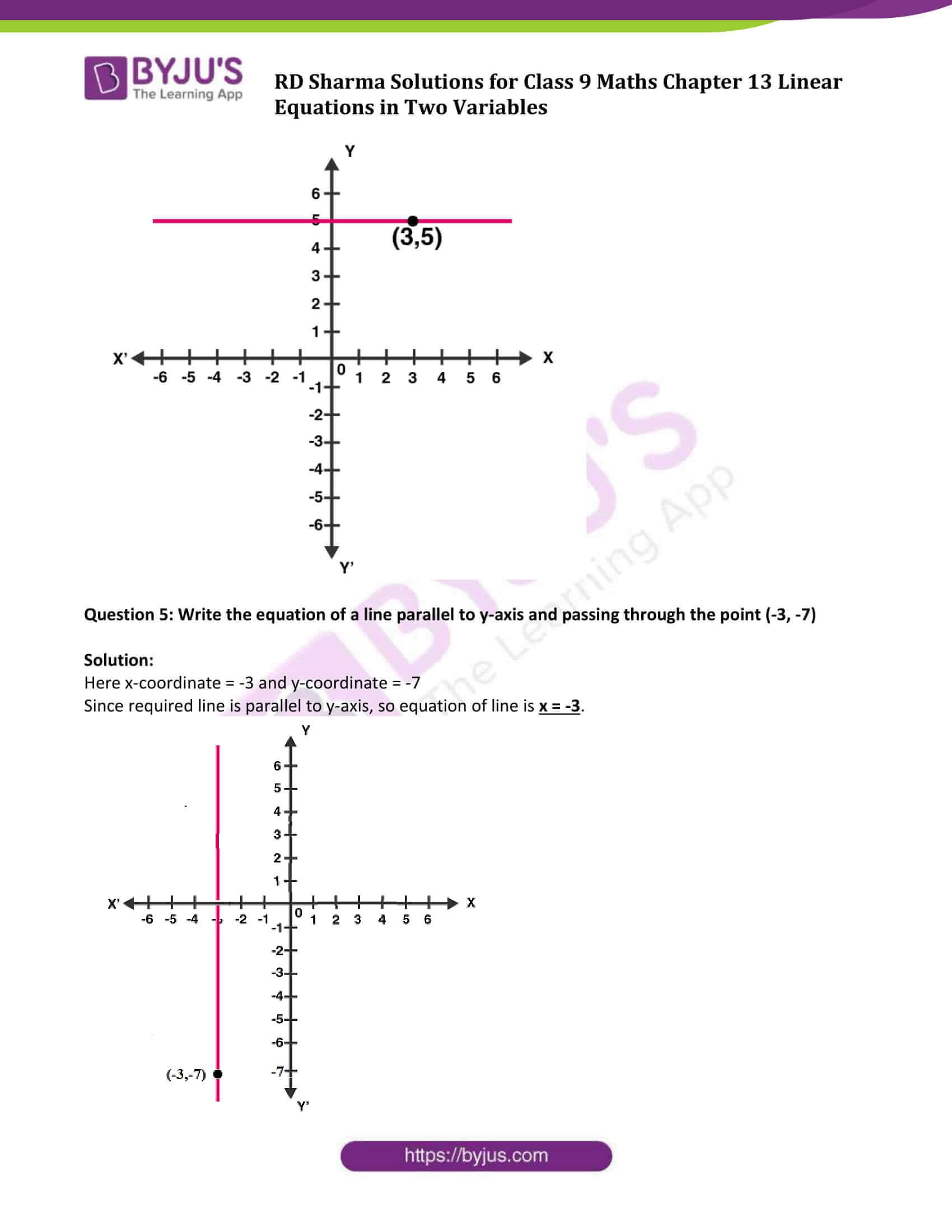### Exercise 13.1

Question 1: Express the following linear equations in the form ax + by + c = 0 and indicate the values of a, b and c in each case:

(i) -2x + 3y = 12 (ii) x – y/2 – 5 = 0 (iii) 2x + 3y = 9.35

(iv) 3x = -7y (v) 2x + 3 = 0 (vi) y – 5 = 0

(vii) 4 = 3x (viii) y = x/2

Solution:

(i) Given equation, -2x + 3y = 12

Or – 2x + 3y – 12 = 0

Comparing the given equation with ax + by + c = 0

We get, a = – 2; b = 3; c = -12

(ii) Given equation, x – y/2 – 5= 0

Comparing the given equation with ax + by + c = 0 ,

We get, a = 1; b = -1/2, c = -5

(iii) Given equation, 2x + 3y = 9.35

or 2x + 3y – 9.35 =0

Comparing the given equation with ax + by + c = 0

We get, a = 2 ; b = 3 ; c = -9.35

(iv) Given equation, 3x = -7y

or 3x + 7y = 0

Comparing the given equation with ax+ by + c = 0,

We get, a = 3 ; b = 7 ; c = 0

(v) Given equation, 2x + 3 = 0

or 2x + 0y + 3 = 0

Comparing the given equation with ax + by + c = 0,

We get, a = 2 ; b = 0 ; c = 3

(vi) Given equation, y – 5 = 0

or 0x + y – 5 = 0

Comparing the given equation with ax + by+ c = 0,

We get, a = 0; b = 1; c = -5

(vii) Given equation, 4 = 3x

or 3x + 0y – 4 = 0

Comparing the given equation with ax + by + c = 0,

We get, a = 3; b = 0; c = -4

(viii) Given equation, y = x/2

Or x – 2y = 0

Or x – 2y + 0 = 0

Comparing the given equation with ax + by + c = 0 ,

We get, a = 1; b = -2; c = 0

Question 2: Write each of the following as an equation in two variables:

(i) 2x = -3 (ii) y=3 (iii) 5x = 7/ 2 (iv) y = 3/2x

Solution:

(i) Given equation, 2x = -3

The above equation can be written in two variables as,

2x + 0y + 3 = 0

(ii) Given equation, y = 3

The above equation can be written in two variables as,

0 x + y – 3 = 0

(iii) Given equation, 5x = 7/2

The above equation can be written in two variables as,

5x + 0y – 7/2 = 0

or 10x + 0y – 7 = 0

(iv) Given equation, y = 3/2 x

The above equation can be written in two variables as,

2y = 3x

3x – 2y = 0

3x – 2y + 0 = 0

Question 3: The cost of ball pen is Rs 5 less than half of the cost of fountain pen. Write this statement as a linear equation in two variables.

Solution:

Let the cost of a fountain pen be y and cost of a ball pen be x.

According to the given statement,

x = y/2 − 5

or 2x = y – 10

or 2x – y + 10 = 0

Which is required linear equation.

### Exercise 13.2

Question 1: Write two solutions for each of the following equations:

(i) 3x + 4y = 7

(ii) x = 6y

(iii) x + πy = 4

(iv) 2/3x – y = 4.

Solution:

(i) 3x + 4y =7 ….(1)

Step 1: Isolate above equation in y.

Subtract 3x from both the sides,

3x + 4y – 3x = 7 – 3x

4y = 7 – 3x

Divide each side by 4

y = 1/4 x (7 – 3x) ….(2)

Step 2: Find Solutions

Substituting x = 1 in (2)

y = 1/4 x (7 – 3) = 1/4 x 4 = 1

Thus x = 1 and y = 1 is the solution of 3x + 4y = 7

Again, Substituting x = 2 in (2)

y = 1/4 x (7 – 3 x 2) = 1/4 x 1 = 1/4

Thus x = 2 and y = 1/4 is the solution of 3x + 4y = 7

Therefore, (1, 1) and (2, 1/4) are two solution of 3x + 4y = 7.

(ii) Given: x = 6y

Substituting x =0 in the given equation,

0 = 6y

or y = 0

Thus (0,0) is one solution

Again, substituting x=6

6 = 6y

or y = 1

Thus, (6, 1) is another solution.

Therefore, (0, 0) and (6, 1) are two solutions of x = 6y.

(iii) Given: x + πy = 4

Substituting x = 0 ⇒ 0 + πy = 4 ⇒ y = 4/ π

Substituting y = 0 ⇒ x + 0 = 4 ⇒ x = 4

Therefore, (0, 4/ π) and (4, 0) are two solutions of x + πy = 4.

(iv) Given: 2/3 x – y = 4

Substituting x = 0 ⇒ 0 – y = 4 ⇒ y = -4

Substituting x = 3 ⇒ 2/3 × 3 – y = 4 ⇒ 2 – y = 4 ⇒ y = -2

Therefore, (0, -4) and (3, -2) are two solutions of 2/3 x – y = 4.

Question 2: Write two solutions of the form x = 0, y = a and x = b, y = 0 for each of the following equations :

(i) 5x – 2y =10

(ii) -4x + 3y =12

(iii) 2x + 3y = 24

Solution:

(i) Given: 5x – 2y = 10

Substituting x = 0 ⇒ 5 × 0 – 2y = 10 ⇒ – 2y = 10 ⇒ – y = 10/2 ⇒ y = – 5

Thus x =0 and y = -5 is the solution of 5x-2y = 10

Substituting y = 0 ⇒ 5x – 2 x 0 = 10 ⇒ 5x = 10 ⇒ x = 2

Thus x =2 and y = 0 is a solution of 5x – 2y = 10

(ii) Given, – 4x + 3y = 12

Substituting x = 0 ⇒ -4 × 0 + 3y = 12 ⇒ 3y = 12 ⇒ y = 4

Thus x = 0 and y = 4 is a solution of the -4x + 3y = 12

Substituting y = 0 ⇒ -4 x + 3 x 0 = 12 ⇒ – 4x = 12 ⇒ x = -3

Thus x = -3 and y = 0 is a solution of -4x + 3y = 12

(iii) Given, 2x + 3y = 24

Substituting x = 0 ⇒ 2 x 0 + 3y = 24 ⇒ 3y =24 ⇒ y = 8

Thus x = 0 and y = 8 is a solution of 2x+ 3y = 24

Substituting y = 0 ⇒ 2x + 3 x 0 = 24 ⇒ 2x = 24 ⇒ x =12

Thus x = 12 and y = 0 is a solution of 2x + 3y = 24

Question 3: Check which of the following are solutions of the equation 2x – y = 6 and which are not:

(i) ( 3 , 0 ) (ii) ( 0 , 6 ) (iii) ( 2 , -2 ) (iv) (√3, 0) (v) (1/2 , -5 )

Solution:

(i) Check for (3, 0)

Put x = 3 and y = 0 in equation 2x – y = 6

2(3) – (0) = 6

6 = 6

True statement.

(3,0) is a solution of 2x – y = 6.

(ii) Check for (0, 6)

Put x = 0 and y = 6 in 2x – y = 6

2 x 0 – 6 = 6

-6 = 6

False statement.

(0, 6) is not a solution of 2x – y = 6.

(iii) Check for (2, -2)

Put x = 0 and y = 6 in 2x – y = 6

2 x 2 – (-2) = 6

4 + 2 = 6

6 = 6

True statement.

(2,-2) is a solution of 2x – y = 6.

(iv) Check for (√3, 0)

Put x = √3 and y = 0 in 2x – y = 6

2 x √3 – 0 = 6

2 √3 = 6

False statement.

(√3, 0) is not a solution of 2x – y = 6.

(v) Check for (1/2, -5)

Put x = 1/2 and y = -5 in 2x – y = 6

2 x (1/2) – (-5) = 6

1 + 5 = 6

6 = 6

True statement.

(1/2, -5) is a solution of 2x – y = 6.

Question 4: If x = -1, y = 2 is a solution of the equation 3x + 4y = k, find the value of k.

Solution:

Given, 3 x + 4 y = k

(-1, 2) is the solution of 3x + 4y = k, so it satisfy the equation.

Substituting x = -1 and y = 2 in 3x + 4y = k, we get

3 (– 1 ) + 4( 2 ) = k

– 3 + 8 = k

k = 5

The value of k is 5.

Question 5: Find the value of λ, if x = –λ and y = 5/2 is a solution of the equation x + 4y – 7 = 0

Solution:

Given, (-λ, 5/2) is a solution of equation 3x + 4y = k

Substituting x = – λ and y = 5/2 in x + 4y – 7 = 0, we get

– λ + 4 (5/2) – 7 =0

-λ + 10 – 7 = 0

λ = 3

Question 6: If x = 2 α + 1 and y = α -1 is a solution of the equation 2x – 3y + 5 = 0, find the value of α.

Solution:

Given, (2 α + 1, α – 1 ) is the solution of equation 2x – 3y + 5 = 0.

Substituting x = 2 α + 1 and y = α – 1 in 2x – 3y + 5 = 0, we get

2(2 α + 1) – 3(α – 1 ) + 5 = 0

4 α + 2 – 3 α + 3 + 5 = 0

α + 10 = 0

α = – 10

The value of α is -10.

Question 7: If x = 1 and y = 6 is a solution of the equation 8x – ay + a^2 = 0, find the values of a.

Solution:

Given, ( 1 , 6 ) is a solution of equation 8x – ay + a^2 = 0

Substituting x = 1 and y = 6 in 8x – ay + a^2 = 0, we get

8 x 1 – a x 6 + a^2 = 0

⇒ a^2 – 6a + 8 = 0 (quadratic equation)

a^2 – 4a – 2a + 8 = 0

a(a – 4) – 2 (a – 4) = 0

(a – 2) (a – 4)= 0

a = 2, 4

Values of a are 2 and 4.

### Exercise 13.3

Question 1: Draw the graph of each of the following linear equations in two variables:

(i) x + y = 4 (ii) x – y = 2 (iii) -x + y = 6

(iv) y = 2x (v) 3x + 5y = 15 (vi) x/2 − y/3 = 2

(vii) (x−2)/3 = y – 3 (viii) 2y = -x +1

Solution:

(i) Given : x + y = 4

or y = 4 – x,

Find values of x and y:

Putting x = 0 ⇒ y = 4

Putting x = 4 ⇒ y = 0

Graph:

Mark points (0, 4) and (4, 0) on the graph and join them.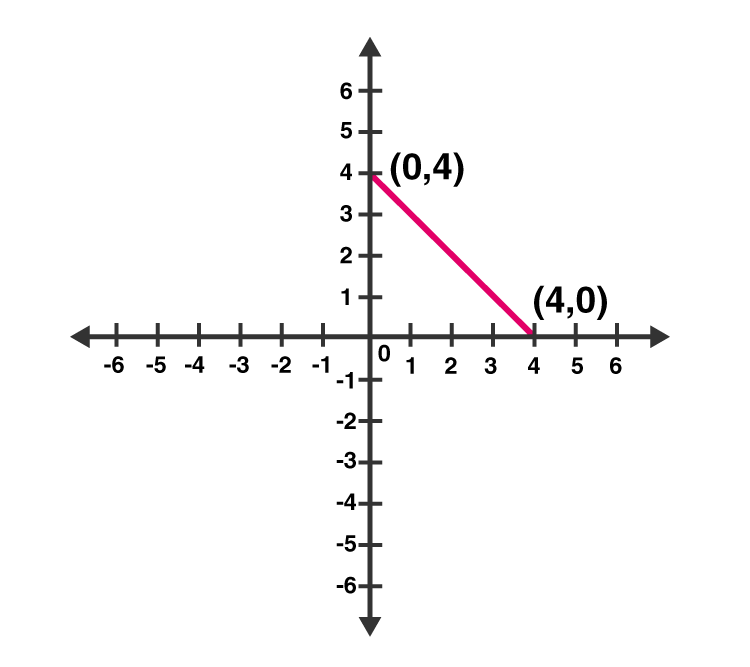(ii) Given: x – y = 2

So, y = x – 2

Putting x = 0 ⇒ y = – 2

Putting x = 2 ⇒ y = 0

Graph:

Mark points (0, -2) and (2, 0) on the graph and join them.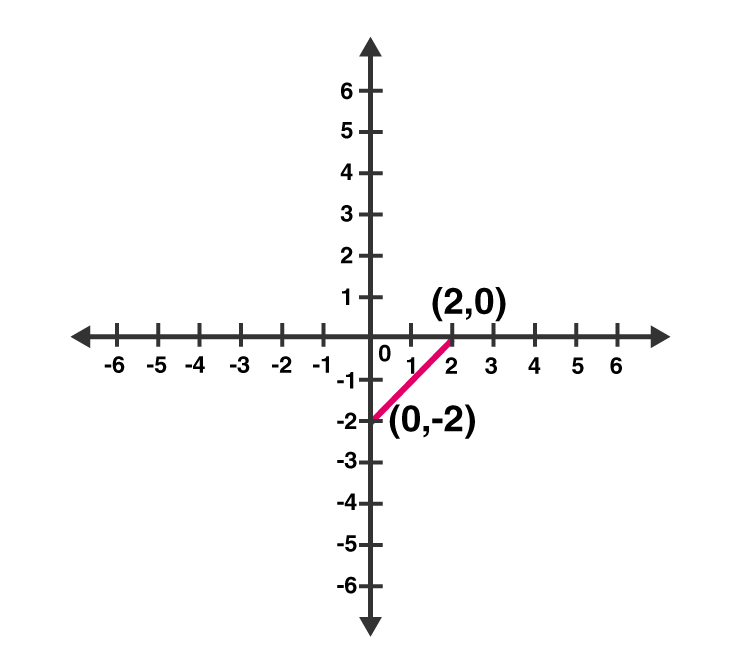(iii) Given: – x + y = 6

So, y = 6 + x

Putting x = 0 ⇒ y =6

Putting x = -6 ⇒ y = 0

Graph:

Mark points (0, 6) and (-6, 0) on the graph and join them.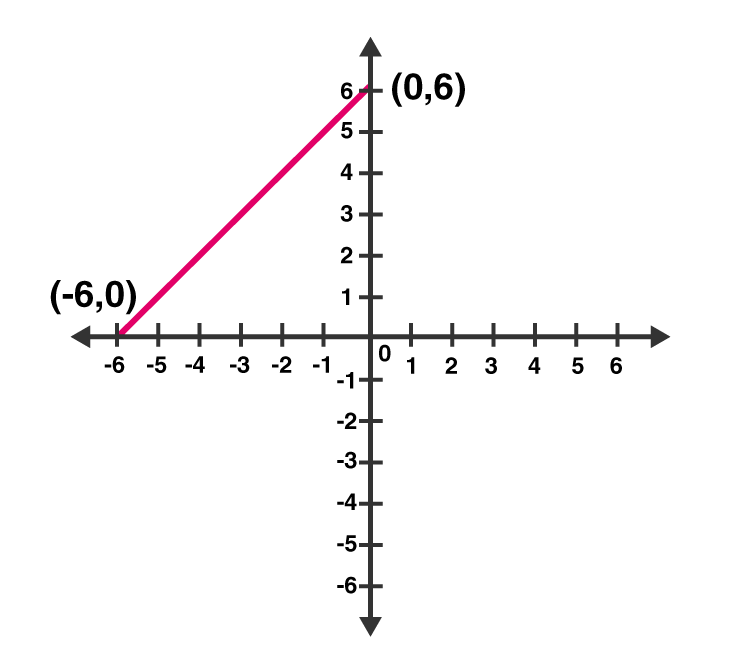(iv) Given: y = 2x

Put x = 1 ⇒ y = 2

Put x = 3 ⇒ y = 6

Graph:

Mark points (1, 2) and (3, 6) on the graph and join them.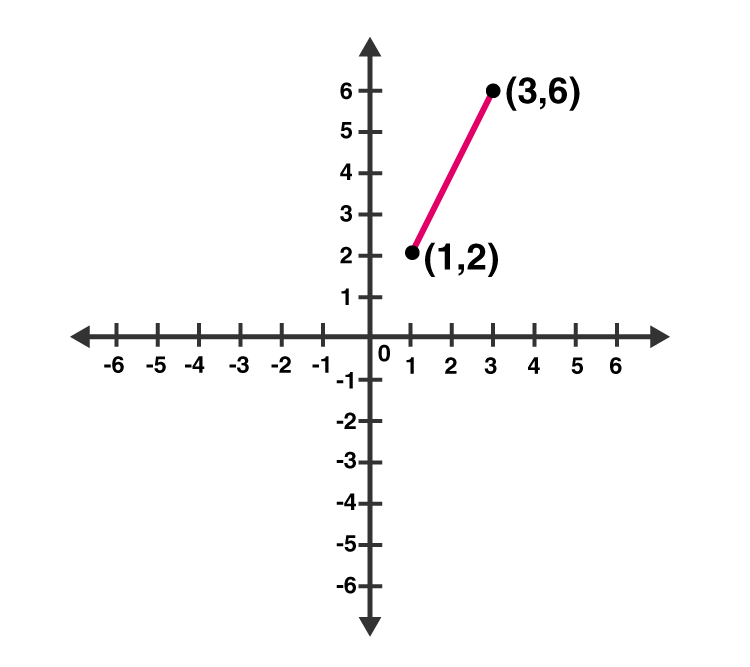(v) Given: 3x + 5y = 15

Or 5y = 15 – 3x

Putting x = 0 ⇒ 5y = 15 ⇒ y =3

Putting x = 5 ⇒ 5y = 0 ⇒ y = 0

Graph:

Mark points (0, 3) and (5, 0) on the graph and join them.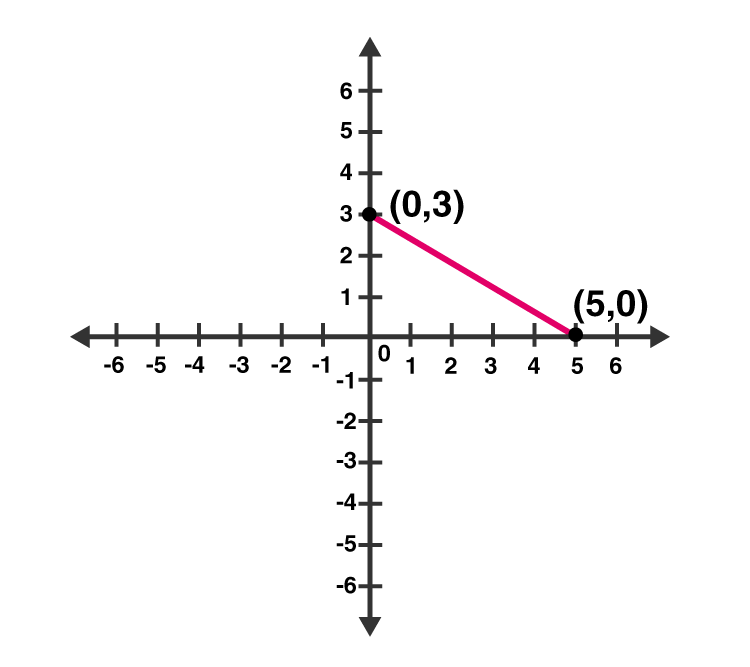(vi) Given: x/2 – y/3 = 2

3x – 2y = 12

y = (3x–12)/2

Putting x = 0 ⇒ y = -6

Putting x = 4 ⇒ y = 0

Graph:

Mark points (0, -6) and (4, 0) on the graph and join them.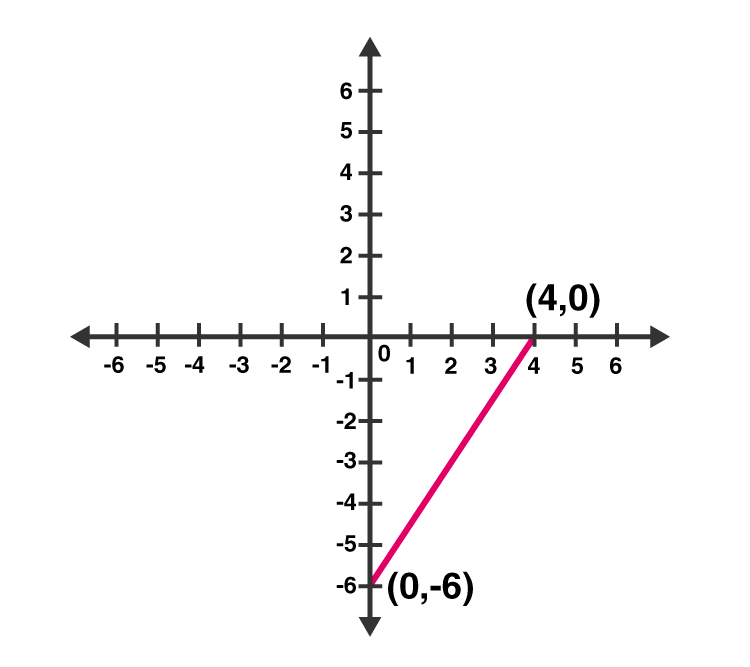(vii) Given: (x −2)/3 = y − 3

x – 2 = 3(y – 3)

x – 2 = 3y – 9

x = 3y – 7

Now, put x = 5 in x = 3y – 7

y = 4

Putting x = 8 in x = 3y – 7,

y = 5

Graph:

Mark points (5, 4) and (8, 5) on the graph and join them.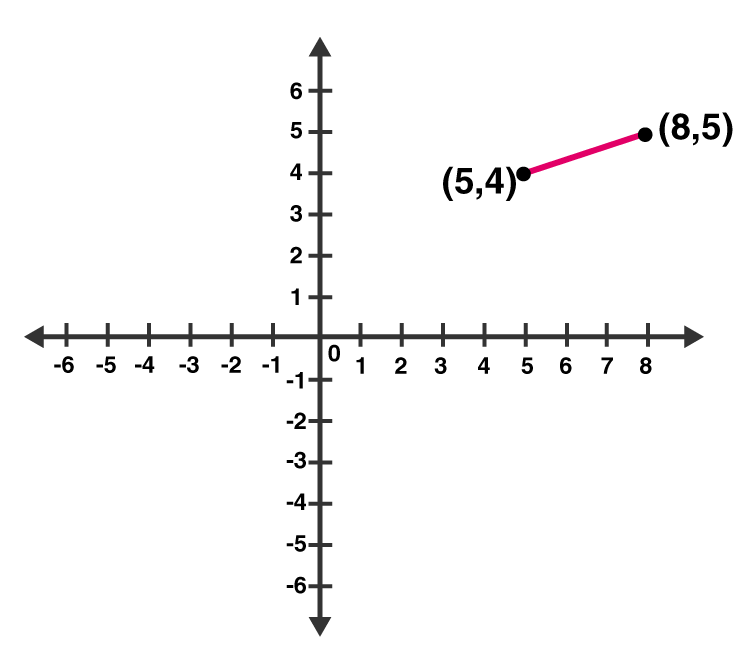(viii) Given: 2y = – x +1

2y = 1 – x

Now, putting x = 1 in 2y = 1 – x, we get;

y = 0

Again, putting x = 5 in 2y = 1 – x, we get;

y = -2

Graph:

Mark points (1, 0) and (5, -2) on the graph and join them.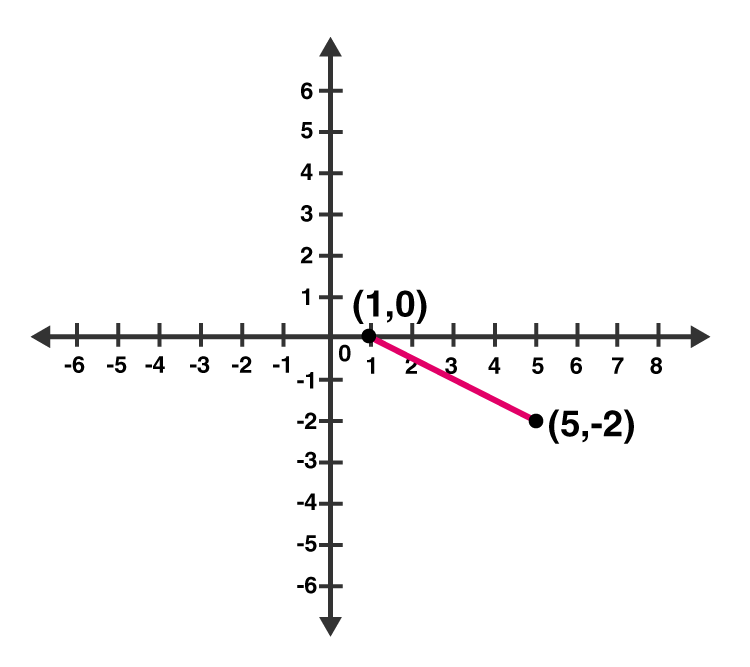Question 2: Give the equations of two lines passing through (3, 12). How many more such lines are there, and why?

Solution:

Since a = 3 and b = 12 is the solution of required equations. So we have to find the set of any two equations which satisfy this point.

Consider 4a – b = 0 and 3a – b + 3 = 0 set of lines which are passing through (3, 12).

We know, infinite lines can be pass through a point.

So, there are infinite lines passing through (3, 12).

Question 3: A three-wheeler scooter charges Rs 15 for first kilometer and Rs 8 each for every subsequent kilometer. For a distance of x km, an amount of Rs y is paid. Write the linear equation representing the above information.

Solution:

Let, total fare for covering the distance of ‘x’ km is given by Rs y

As per the given statement;

y = 15 + 8(x – 1)

y = 15 + 8x – 8

y = 8x + 7

Above equation represents the linear equation for the given information.

Question 4: A lending library has a fixed charge for the first three days and an additional charge for each day thereafter. Aarushi paid Rs 27 for a book kept for seven days. If fixed charges are Rs x and per day charges are Rs y. Write the linear equation representing the above information.

Solution:

Aarushi paid Rs 27, of which Rs. x for the first three days and Rs. y per day for 4 more days is given by

x + (7 – 3) y = 27

x + 4y = 27

Above equation represents the linear equation for the given information.

Question 5: A number is 27 more than the number obtained by reversing its digits. lf its unit’s and ten’s digit are x and y respectively, write the linear equation representing the statement.

Solution:

Given: The original number is 27 more than the number obtained by reversing its digits

The given number is in the form of 10y + x.

Number produced by reversing the digits of the number is 10x + y.

As per statement:

10y + x = 10x + y + 27

10y – y + x – 10x = 27

9y – 9x = 27

9 (y – x) = 27

y – x = 3

x – y + 3 = 0

Above equation represents the required linear equation.

Question 6: The Sum of a two digit number and the number obtained by reversing the order of its digits is 121. If units and ten’s digit of the number are x and y respectively, then write the linear equation representing the above statement.

Solution:

As per the statement given, the number is 10y + x.

On reversing the digits of the number, we get, 10x + y

Sum of the two numbers is 121. (Given)

10y + x + 10x + y = 121

11x + 11y = 121

x + y = 11

Which represents the required linear equation.

Question 7: Plot the Points (3, 5) and (-1, 3) on a graph paper and verify that the straight line passing through the points, also passes through the point (1, 4).

Solution:

Plot points (3, 5), (-1, 3) and (1, 4) on a graph.

Let A(1, 4), B(3, 5) and C(-1, 3)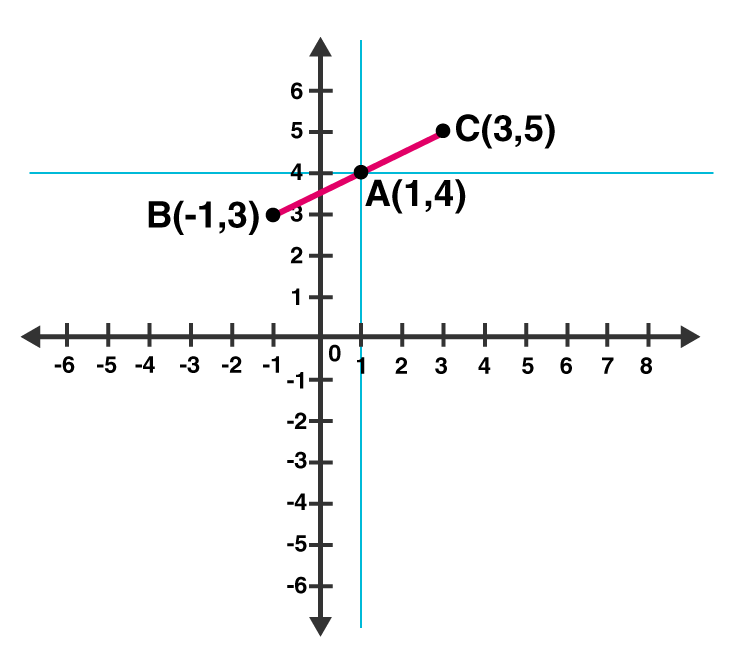From above graph, we can see that, Point A (1, 4) is already plotted on the graph, and a point of intersection of two intersecting lines.

Hence, it is proved that the straight line passing through (3, 5) and (-1, 3) and also passes through A (1, 4).

Question 8: From the choices given below, choose the equations whose graph is given in figure.

(i) y = x (ii) x + y = 0 (iii) y = 2x (iv) 2 + 3y = 7x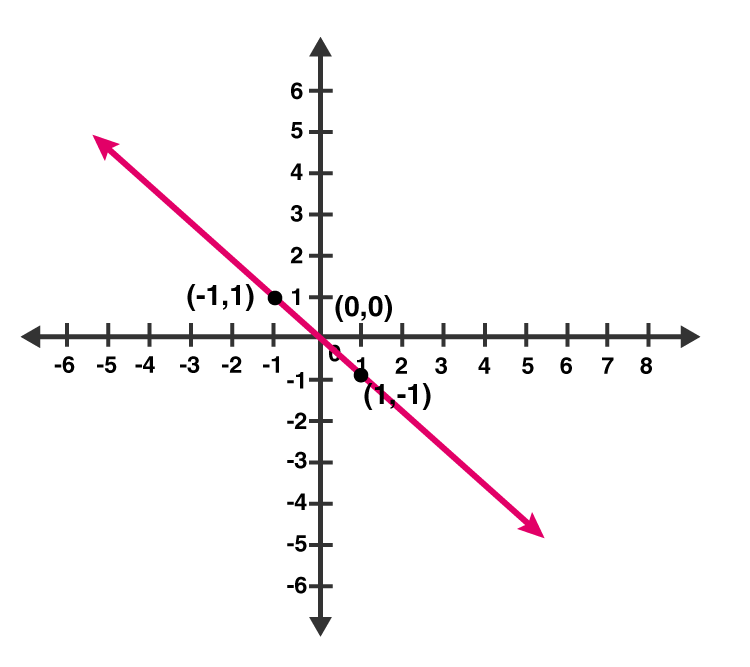Solution:

From graph, co-ordinates (1, -1) and (-1, 1) are solutions of one of the equations.

We will put the value of all the co-ordinates in each equation and check which equation satisfy them.

(i) y = x

Put x = 1 and y = -1 ,

Thus, 1 ≠ -1

L.H.S ≠ R.H.S

Putting x = -1 and y = 1 ,

-1 ≠ 1

L.H.S ≠ R.H.S

Therefore, y = x does not represent the graph in the given figure.

(ii) x + y = 0

Putting x = 1 and y = -1 ,

⇒ 1 + (-1) = 0

⇒ 0 = 0

L.H.S = R.H.S

Putting x = -1 and y = 1 ,

(-1) + 1 = 0

0 = 0

L.H.S = R.H.S

Thus, the given solutions satisfy this equation.

(iii) y = 2x

Putting x = 1 and y = -1

-1 = 2 (Not True)

Putting x = -1 and y = 1

1 = -2 (Not True)

Thus, the given solutions does not satisfy this equation.

(iv) 2 + 3y = 7x

Putting x = 1 and y = -1

2 – 3 = 7

-1 = 7 (Not true)

Putting x = -1 and y = 1

2 + 3 = -7

5 = -7 (Not True)

Thus, the given solutions does not satisfy this equation.

Question 9: From the choices given below, choose the equation whose graph is given fig:

(i) y = x + 2 (ii) y = x – 2 (iii)y = – x + 2 (iv) x + 2y = 6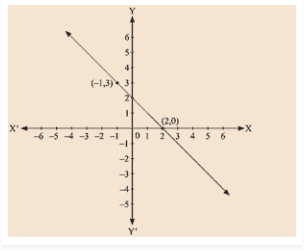Solution:

Given: (-1, 3) and (2, 0) are the solution of one of the following given equations.

Check which equation satisfy both the points.

(i) y = x + 2

Putting, x = -1 and y = 3

3 ≠ – 1 + 2

L.H.S ≠ R.H.S

Putting, x = 2 and y = 0

0 ≠ 4

L.H.S ≠ R.H.S

Thus, this solution does not satisfy the given equation.

(ii) y = x – 2

Putting, x = -1 and y = 3

3 ≠ – 1 – 2

L.H.S ≠ R.H.S

Putting, x = 2 and y = 0

0 = 0

L.H.S = R.H.S

Thus, the given solutions does not satisfy this equation completely.

(iii) y = – x + 2

Putting, x = – 1 and y = 3

3 = – ( – 1 ) + 2

L.H.S = R.H.S

Putting x = 2 and y = 0

0 = -2 + 2

0 = 0

L.H.S = R.H.S

Therefore, (0, 2) and (-1,3) satisfy this equation.

Hence, this is the graph for equation y = -x + 2 .

(iv) x + 2y = 6

Putting, x = – 1 and y = 3

-1 + 2(3) = 6

-1 + 6 = 6

5 = 6

L.H.S ≠ R.H.S

Putting x = 2 and y = 0

2 + 2(0) = 6

2 = 6

L.H.S ≠ R.H.S

Thus, this solution does not satisfy the given equation.

Question 10 : If the point (2, -2) lies on the graph of linear equation, 5x + ky = 4, find the value of k.

Solution:

Point (2,-2) lies on the given linear equation, which implies (2, -2) satisfy this equation 5x + ky = 4.

Now, putting x = 2 and y = -2 in 5x + ky = 4

5 × 2 + ( -2 ) k = 4

10 – 2k = 4

2k = 10 – 4

2k = 6

k = 6/2 = 3

The value of k is 3.

### Exercise 13.4

Question 1: Give the geometric representations of the following equations

(a) on the number line (b) on the Cartesian plane:

(i) x = 2 (ii) y + 3 = 0 (iii) y = 3 (iv) 2x + 9 = 0 (v) 3x – 5 = 0

Solution:

(i) x = 2

The representation of equation on the number line: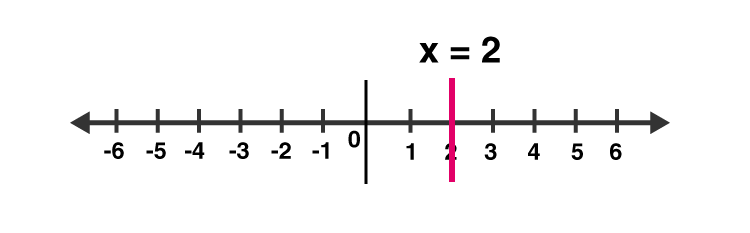The representation of equation on the Cartesian plane: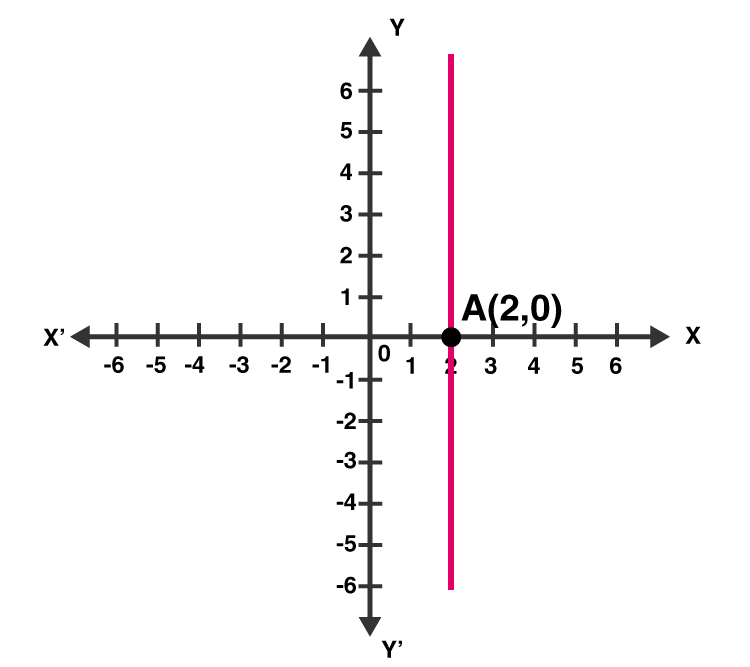(ii) y + 3 = 0

or y = -3

The representation of equation on the number line: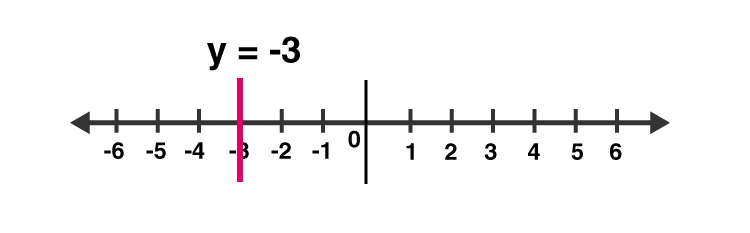The representation of equation on the Cartesian plane: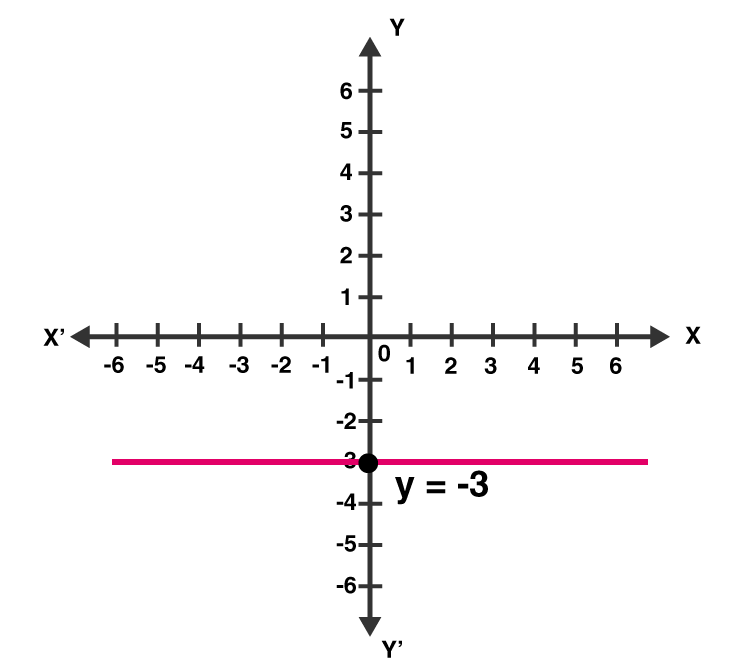(iii) y = 3

The representation of equation on the number line: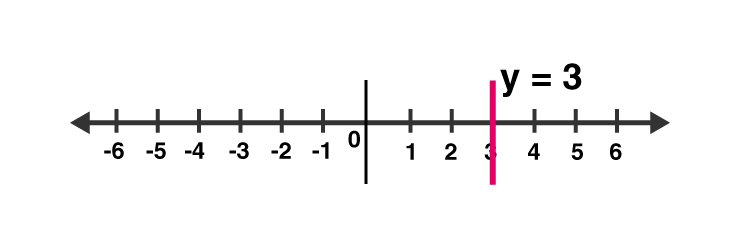The representation of equation on the Cartesian plane: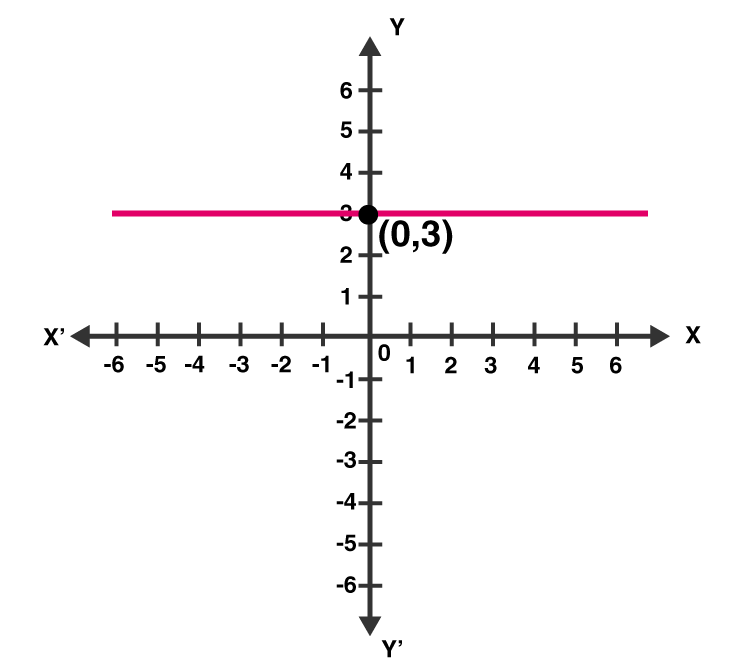(iv) 2x + 9 = 0

or x = -9/2

The representation of equation on the number line: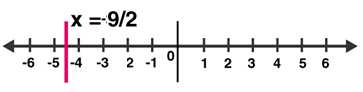The representation of equation on the Cartesian plane: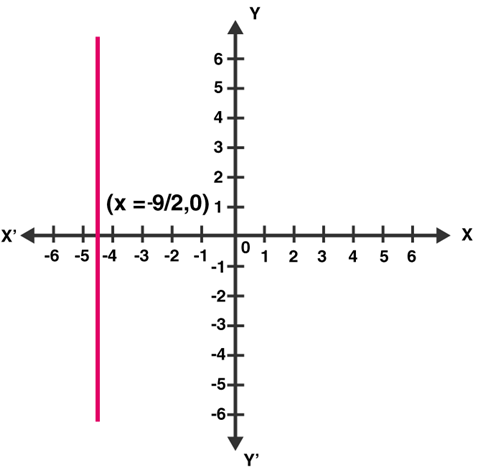(v) 3x – 5 = 0

or x = 5/3

The representation of equation on the number line: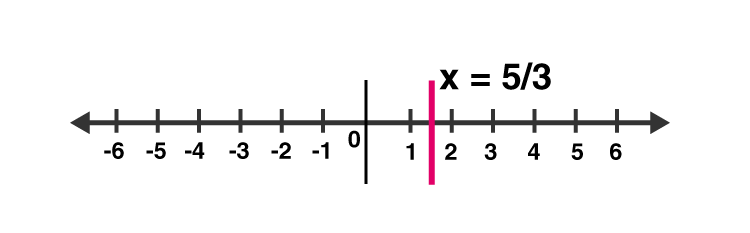The representation of equation on the Cartesian plane: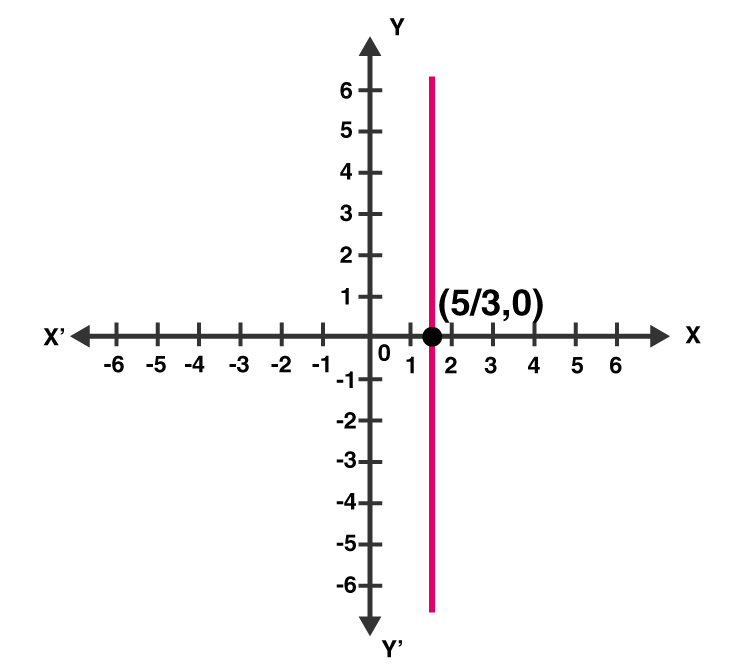Question 2 : Give the geometrical representation of 2x + 13 = 0 as an equation in

(i) one variable (ii) two variables

Solution:

2x + 13 = 0

(i) Isolate given equation in x

Subtract 13 from both the sides

2x + 13 – 13 = 0 – 13

2x = -13

Divide each side by 2

x = -13/2 = -6.5

Which is an equation in one variable.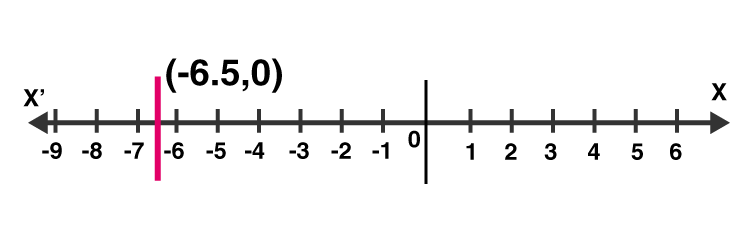(ii) 2x + 13 = 0 can be written as 2x + 0y + 13 = 0

The representation of the solution on the Cartesian plane: A line parallel to y axis passing through the point (-13/2 , 0):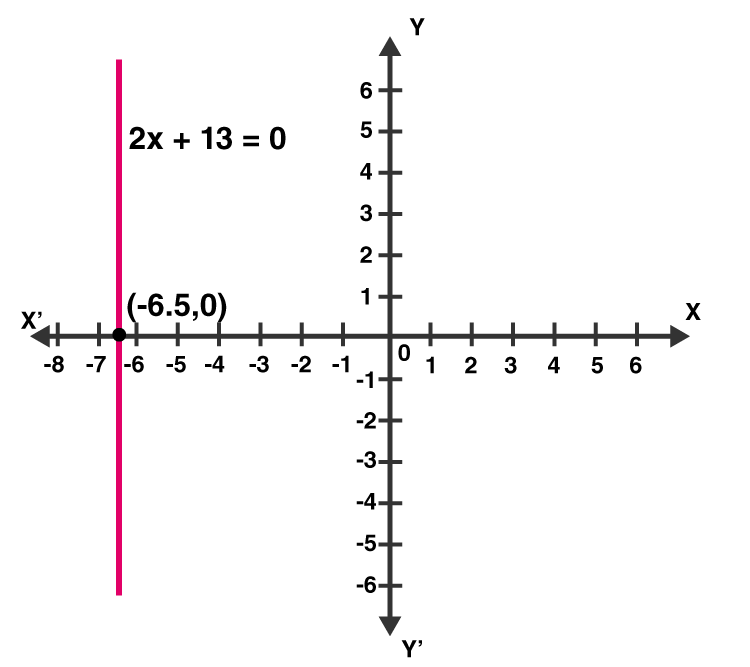### Exercise VSAQs Page No: 13.32

Question 1: Write the equation representing x-axis.

Solution: y = 0

Question 2: Write the equation representing y-axis.

Solution: x = 0

Question 3: Write the equation of a line passing through the point (0, 4) and parallel to x-axis.

Solution: Here, x-coordinate is zero and y-coordinate is 4, so equation of line passing through the point (0, 4) is y = 4.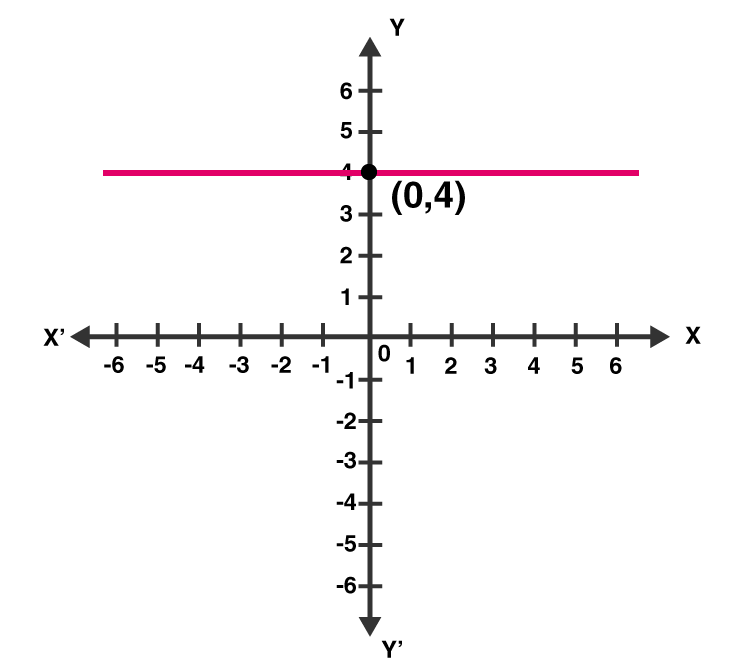Question 4: Write the equation of a line passing through the point (3, 5) and parallel to x-axis.

Solution: Here x-coordinate = 3 and y-coordinate = 5

Since required line is parallel to x-axis, so equation of line is y = 5.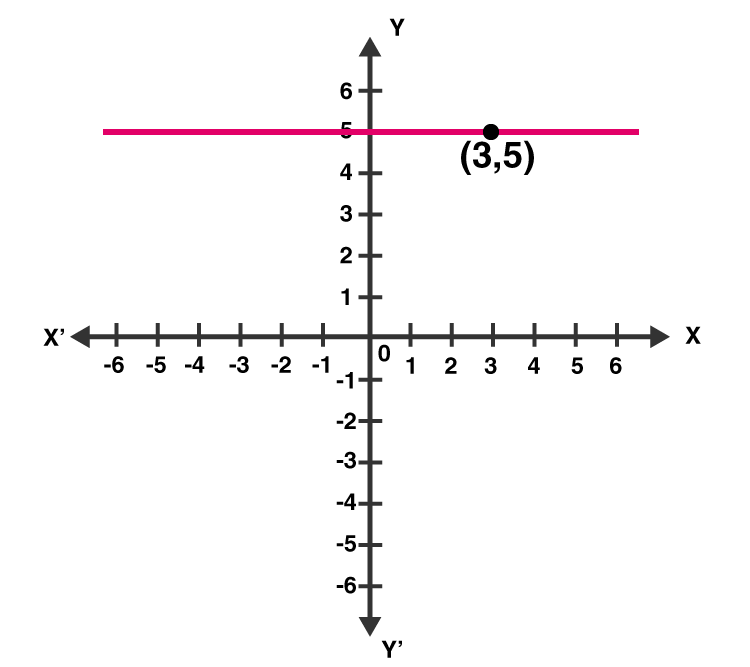Question 5: Write the equation of a line parallel to y-axis and passing through the point (-3, -7)

Solution:

Here x-coordinate = -3 and y-coordinate = -7

Since required line is parallel to y-axis, so equation of line is x = -3.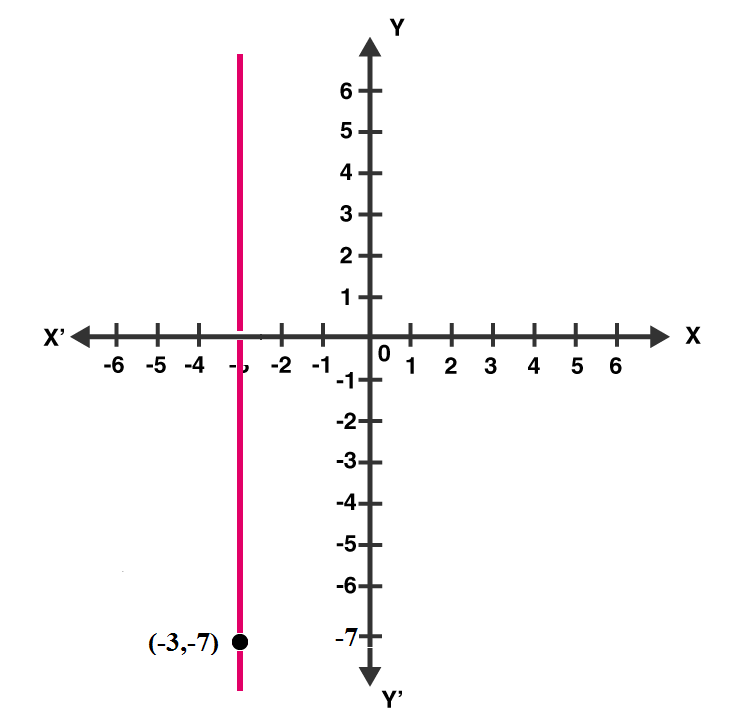## RD Sharma Solutions for Class 9 Maths Chapter 13 Linear Equations in Two Variables

In this 13th Chapter of Class 9 RD Sharma Solutions students will study important concepts listed below:

• Linear Equations in Two Variables Introduction.
• Solution of a linear equation.
• Graph of a linear equation in two variables.
• Equations of lines parallel to the x-axis and y-axis.

## Frequently Asked Questions on RD Sharma Solutions for Class 9 Maths Chapter 13

### Where can I get the precise answers of RD Sharma Solutions for Class 9 Maths Chapter 13?

Students can find the accurate answers to each question of RD Sharma Solutions for Class 9 Maths Chapter 13 at BYJU’S. The solutions of the RD Sharma textbook are developed by Mathematics experts in an efficient manner. These solutions are so apt that students who follow these solutions while revising the textbook problems obtain skills essential from an exam perspective.

### Name the topics discussed in RD Sharma Solutions for Class 9 Maths Chapter 13

The topics covered in RD Sharma Solutions for Class 9 Maths Chapter 13 are given below:

• Linear Equations in Two Variables Introduction.
• Solution of a linear equation.
• Graph of a linear equation in two variables.
• Equations of lines parallel to the x-axis and y-axis.

### Is it necessary to follow RD Sharma Solutions for Class 9 Maths Chapter 13 while solving textbook problems?

Yes, students who practice diligently RD Sharma Solutions for Class 9 Maths Chapter 13 while solving textbook problems obtain a thorough knowledge of concepts. These solutions are curated by knowledgeable teachers as per the current CBSE syllabus. Each solution of the textbook is presented in a clear format to help students understand the concept in a detailed manner and procure more marks in board exams.